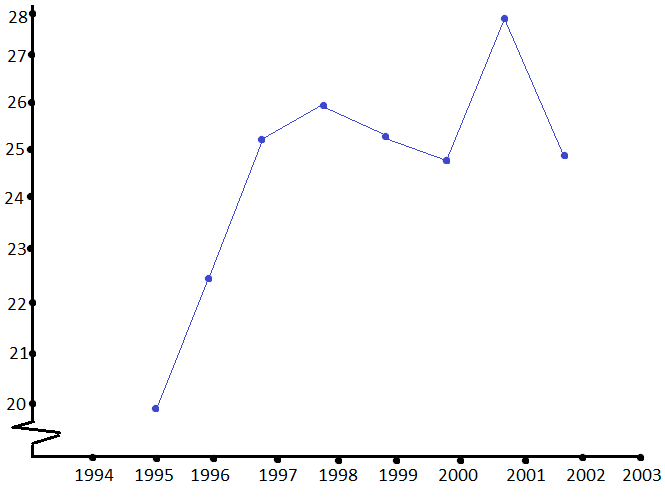ISC Class 11 Maths Sample Question Paper – Mock 1 with Answers are provided as per the board syllabus and pattern at BYJU’S. These mock sample papers of Class 11 ISC can be downloaded in the form of a pdf and also can be accessed online. These papers cover the entire syllabus of ISC Maths Class 11 and are provided by considering the weightage for each concept.

Practice is the best key for the students who wish to score full marks for the questions of maths in their board examination. This can be achieved by practising more sample papers of Maths Class 11 ISC. Students can download these papers and they can also verify their answers after solving them.

## ISC Class 11 Maths Mock Sample Question Paper 1 with Solutions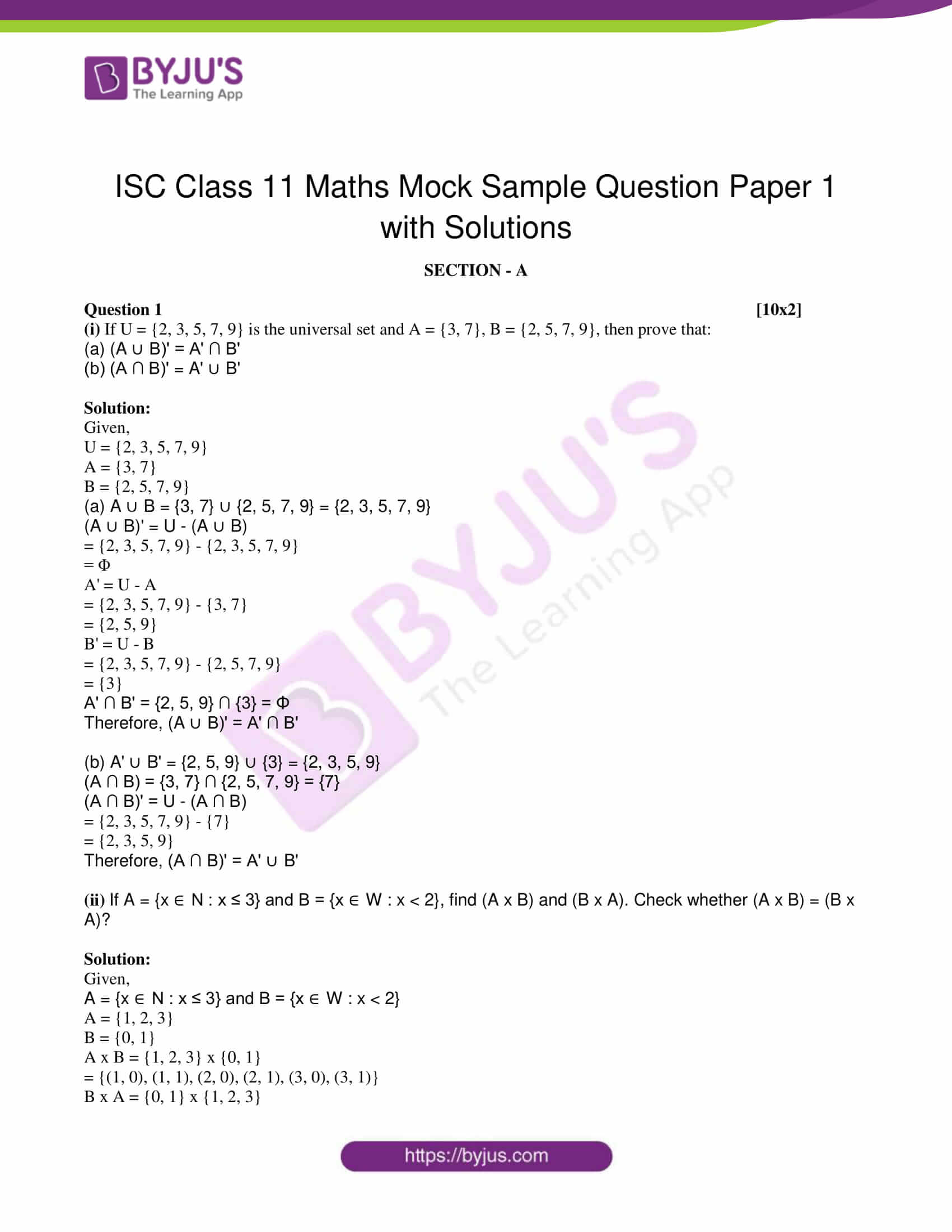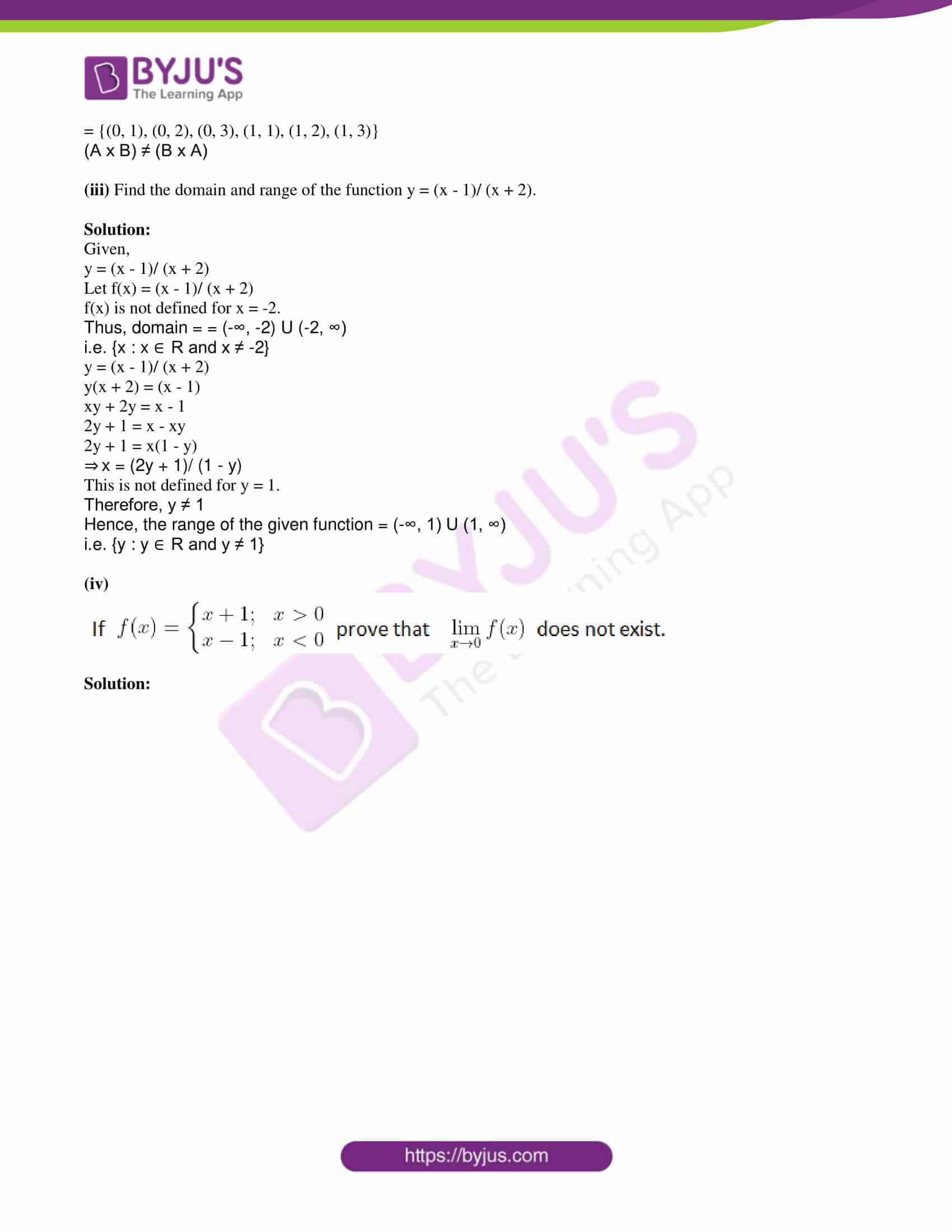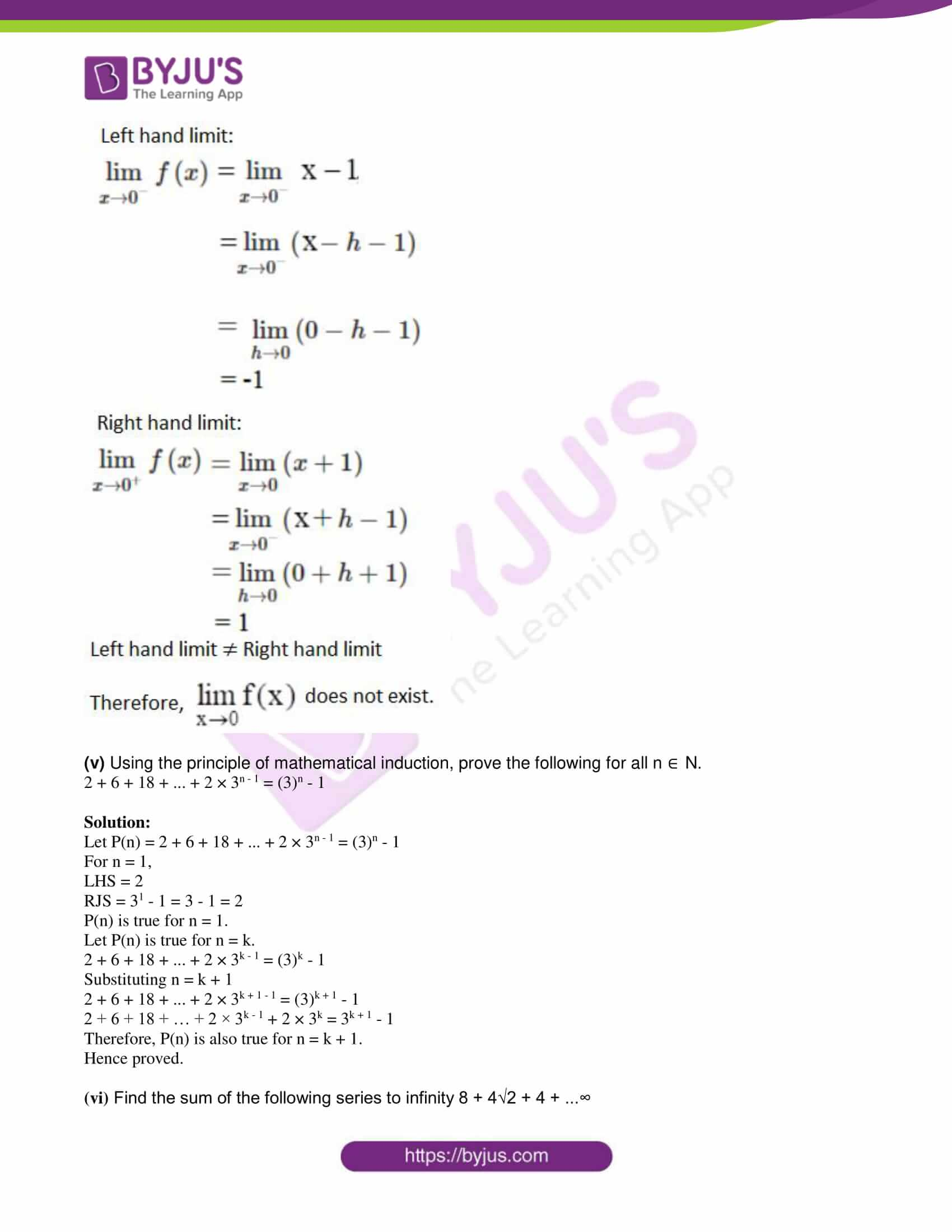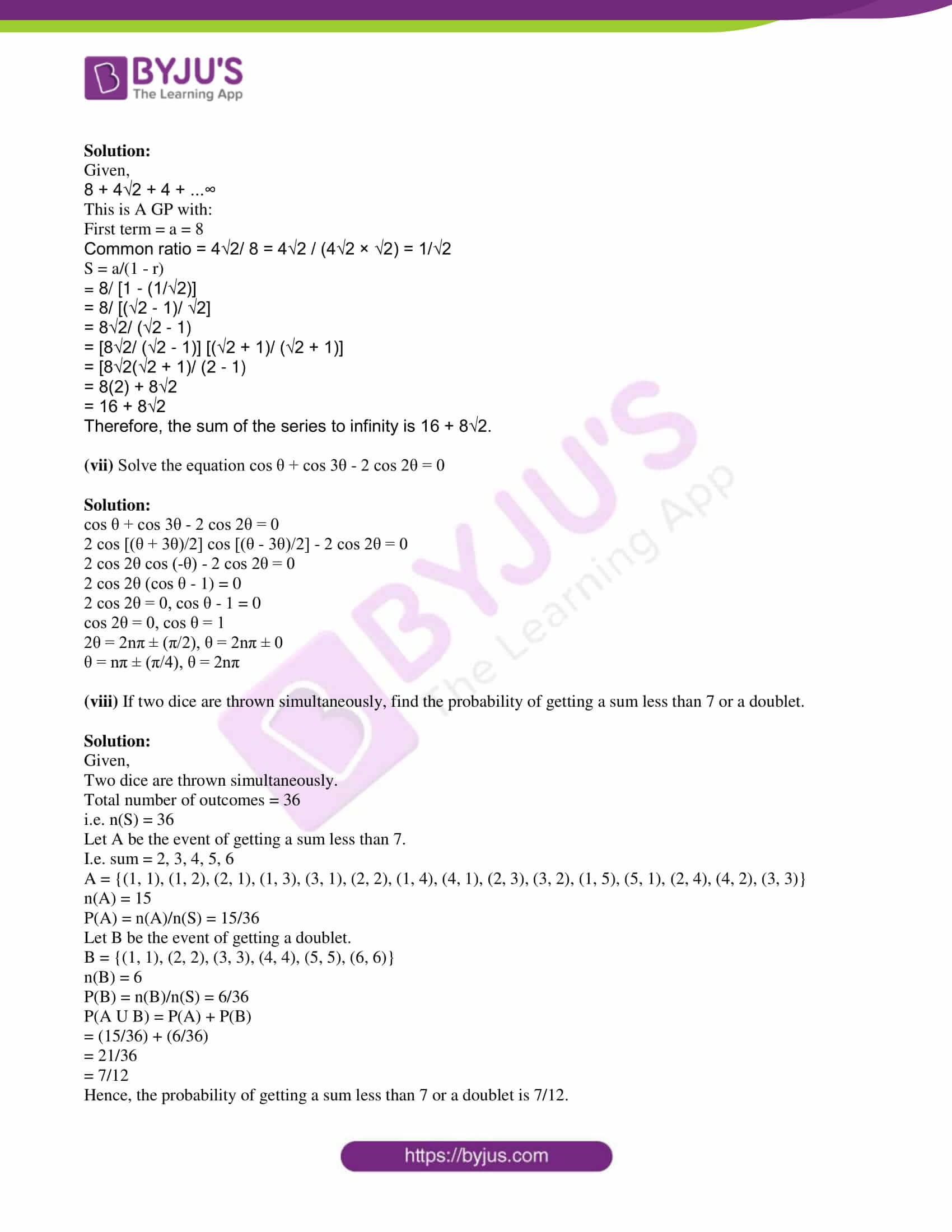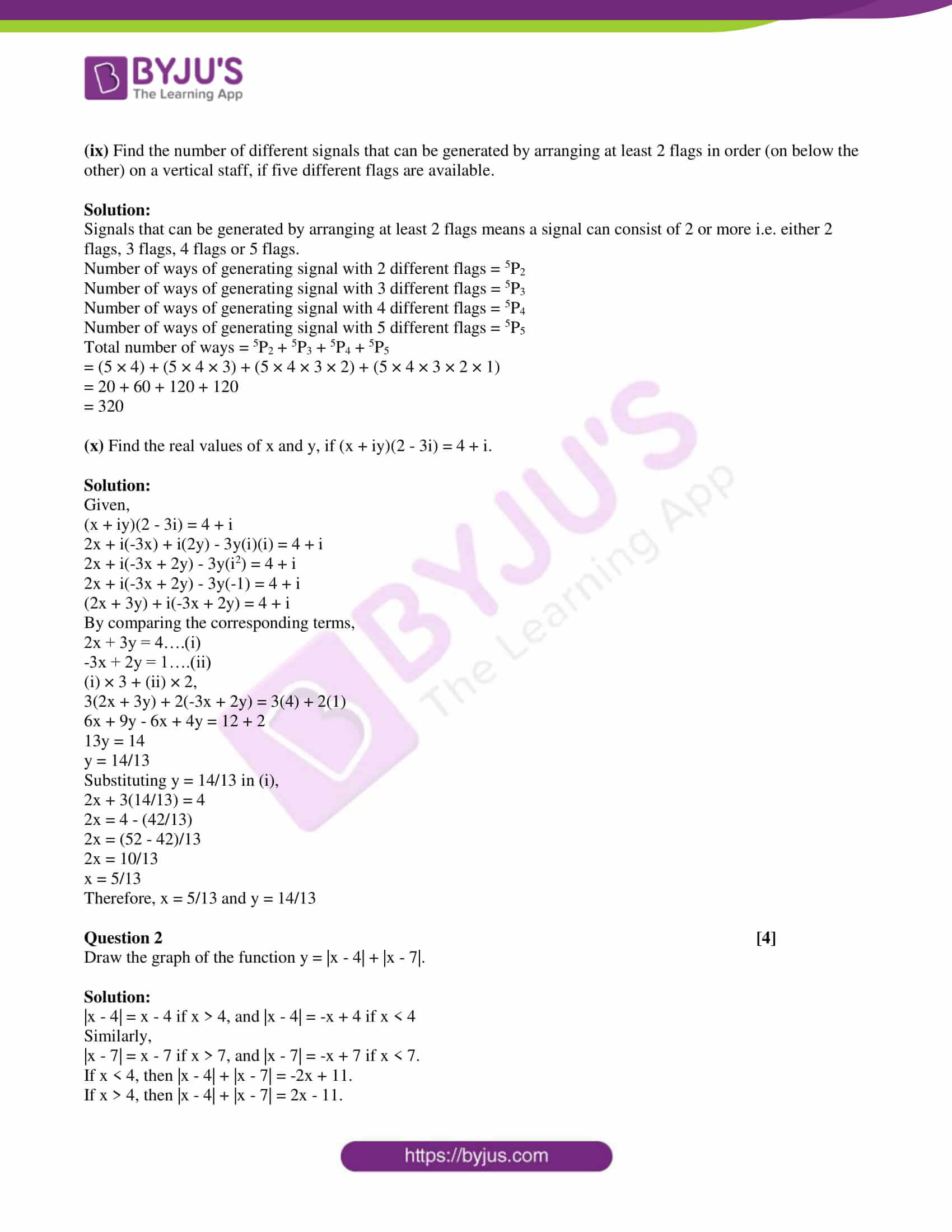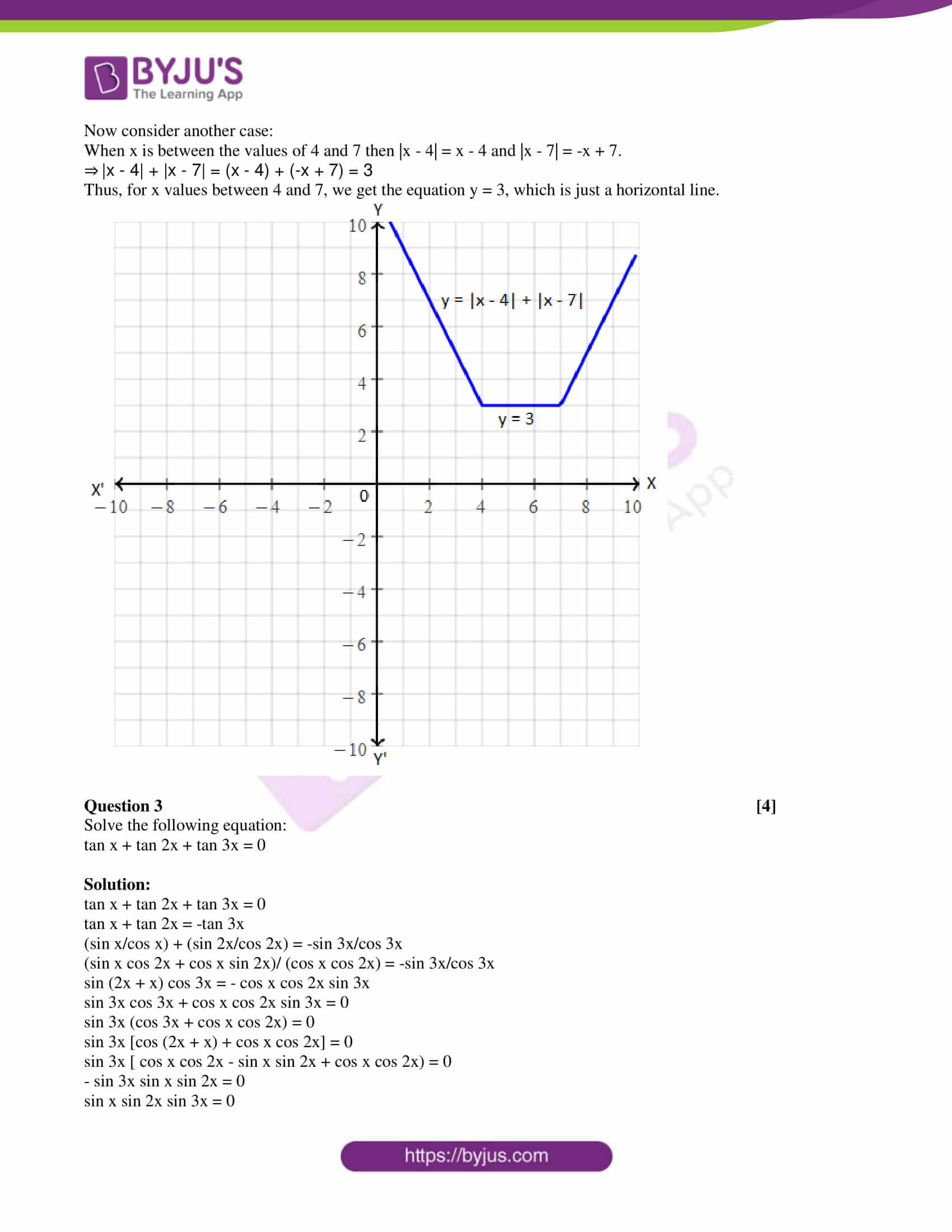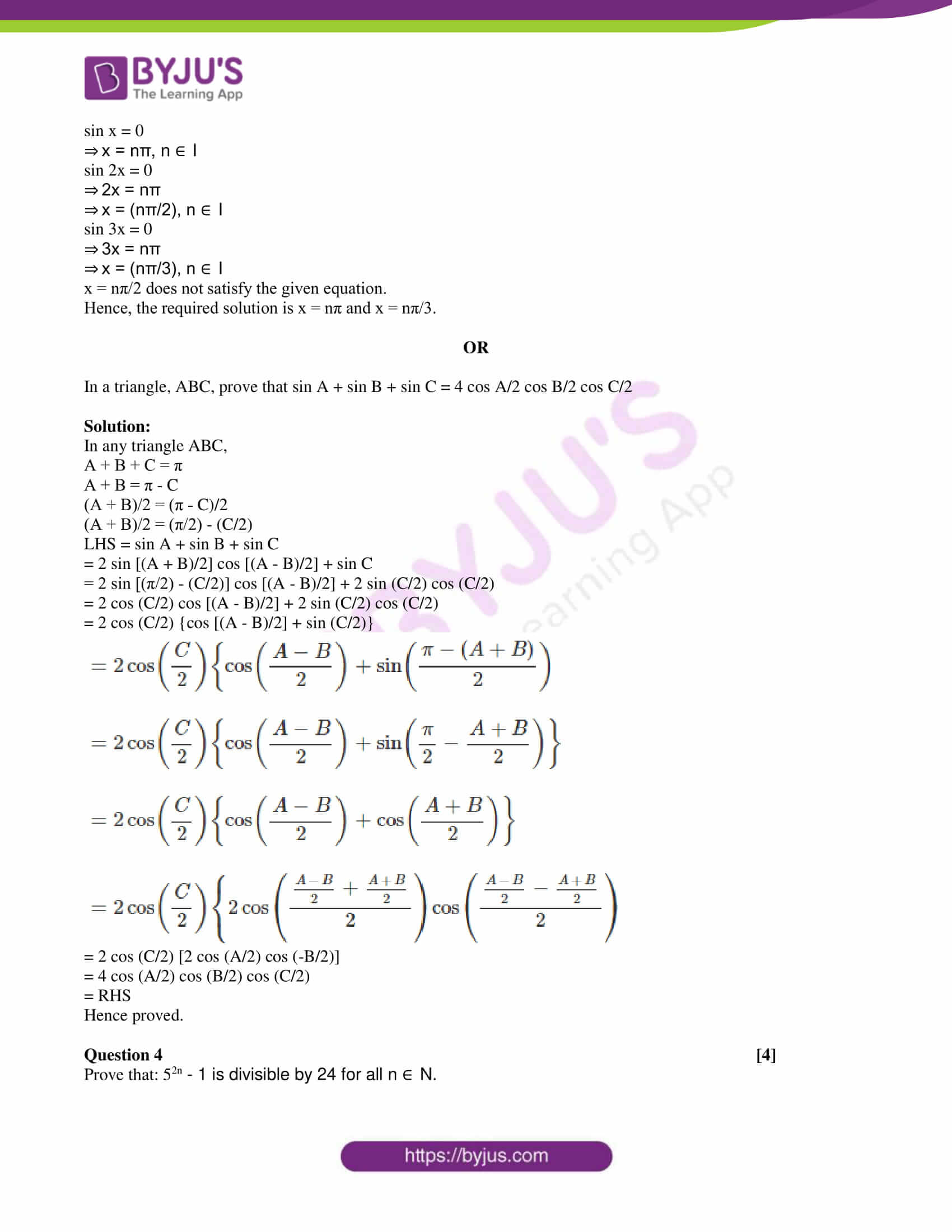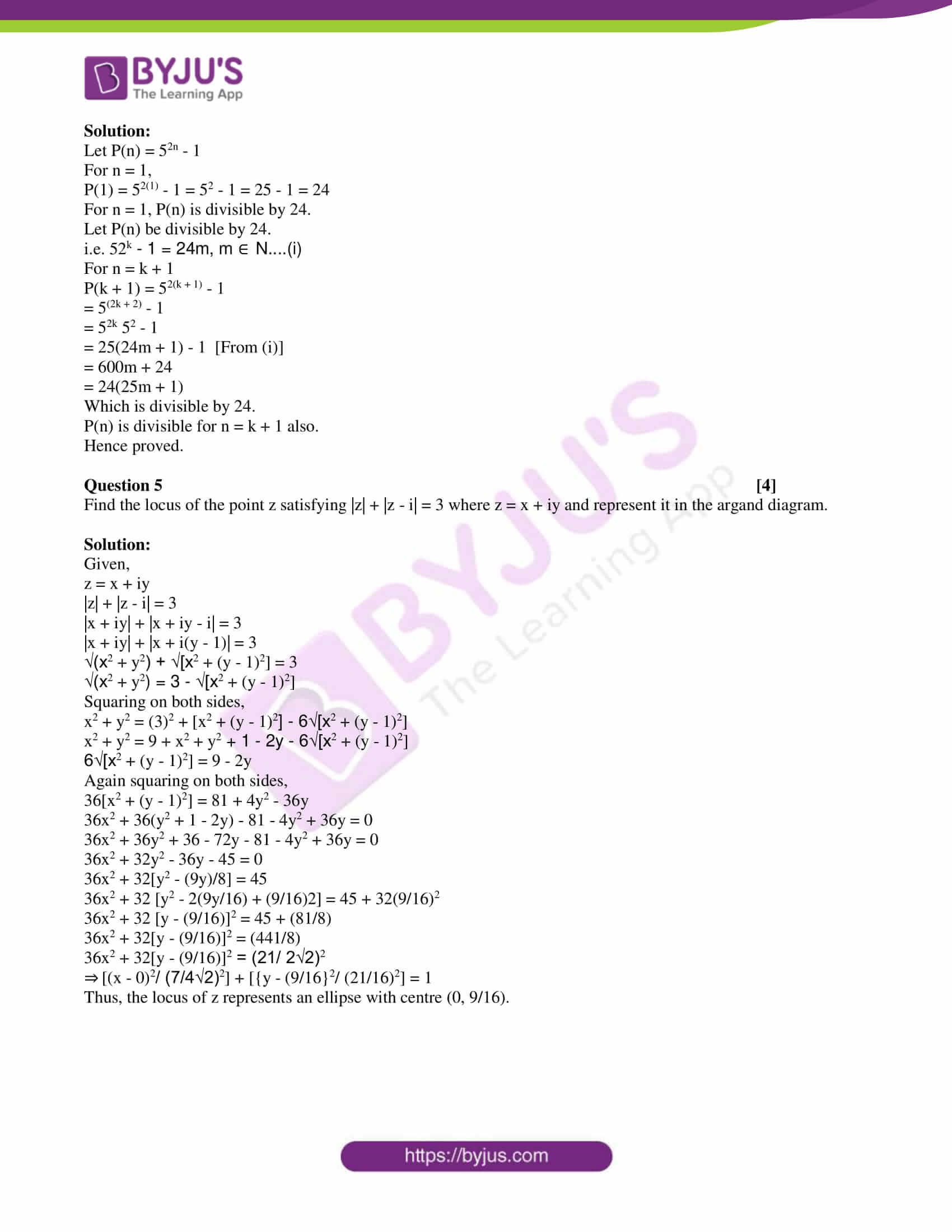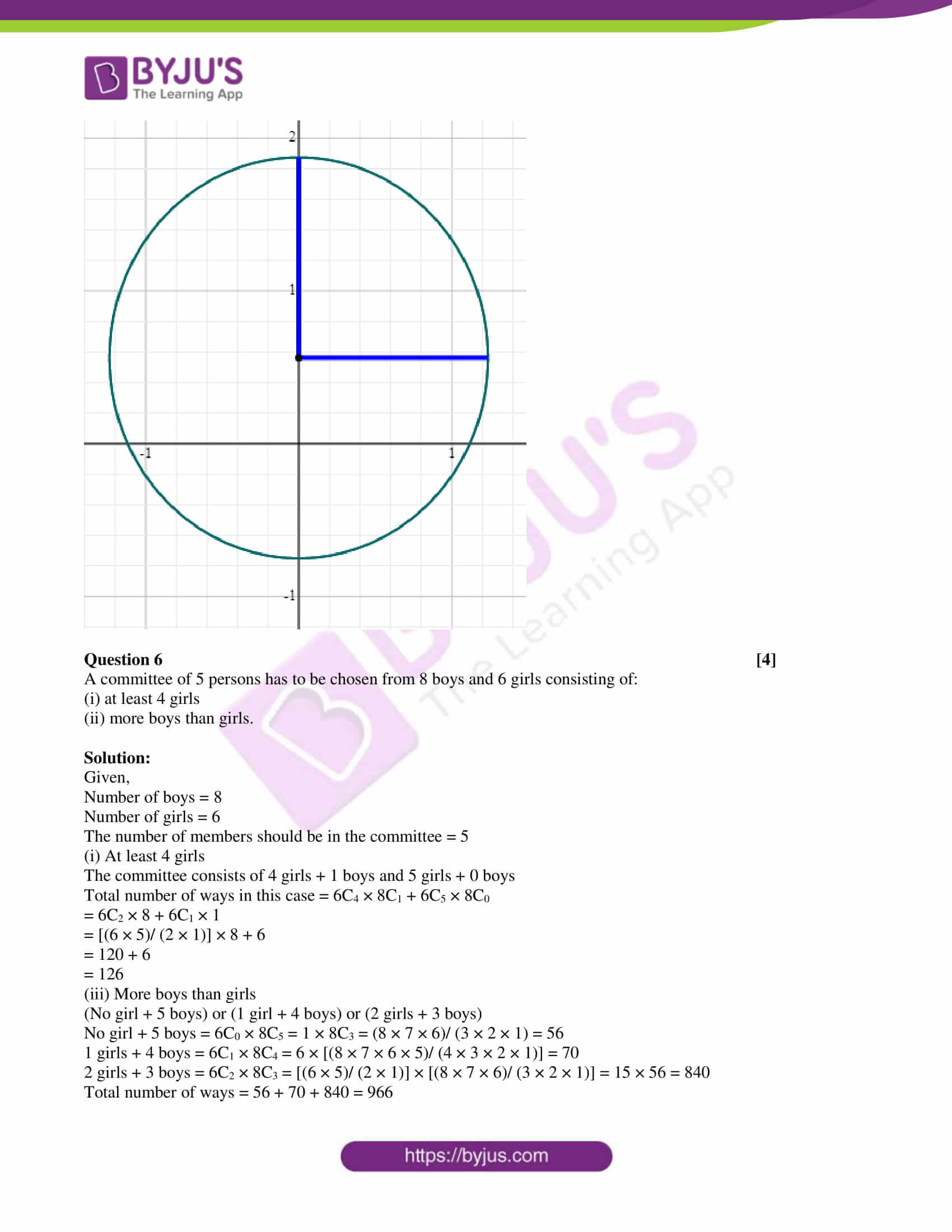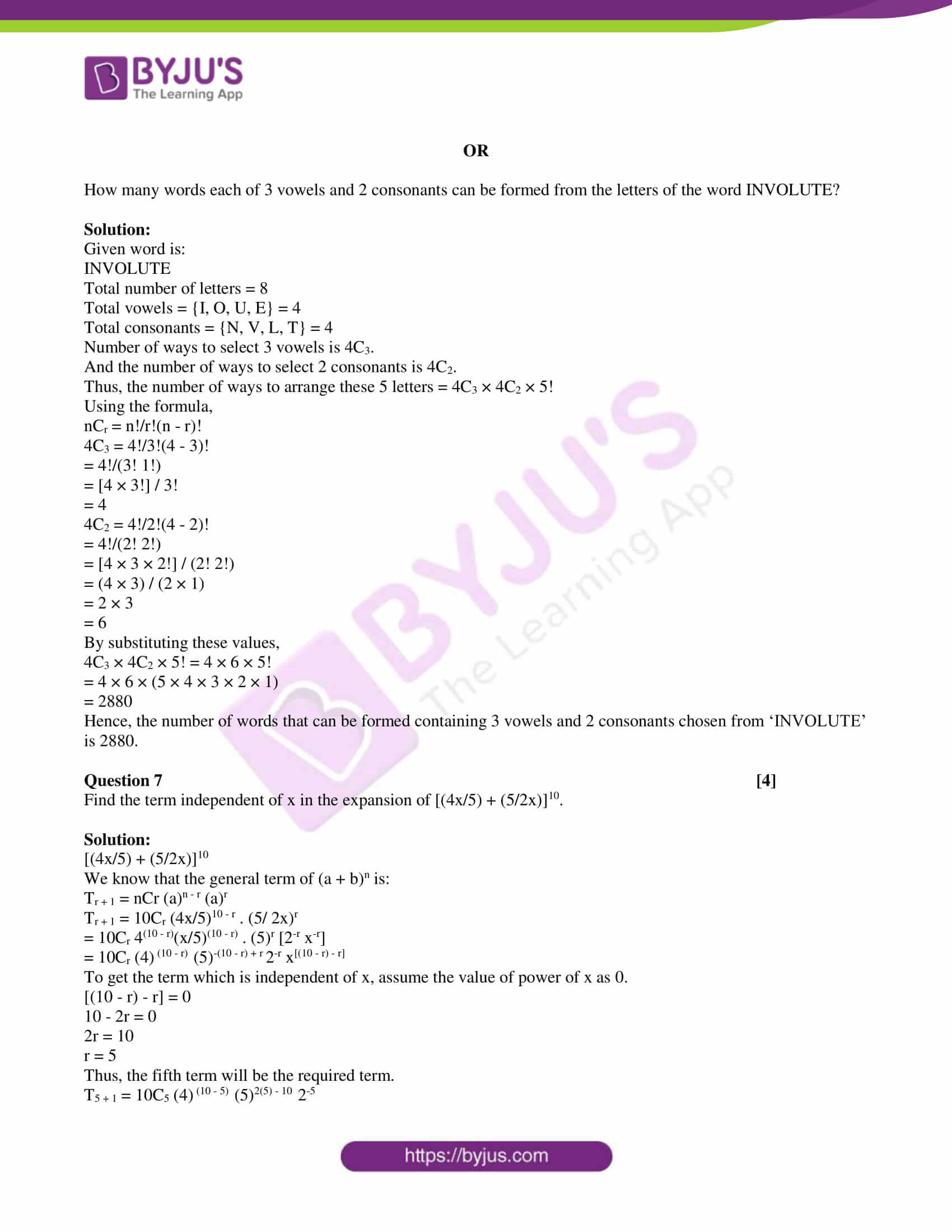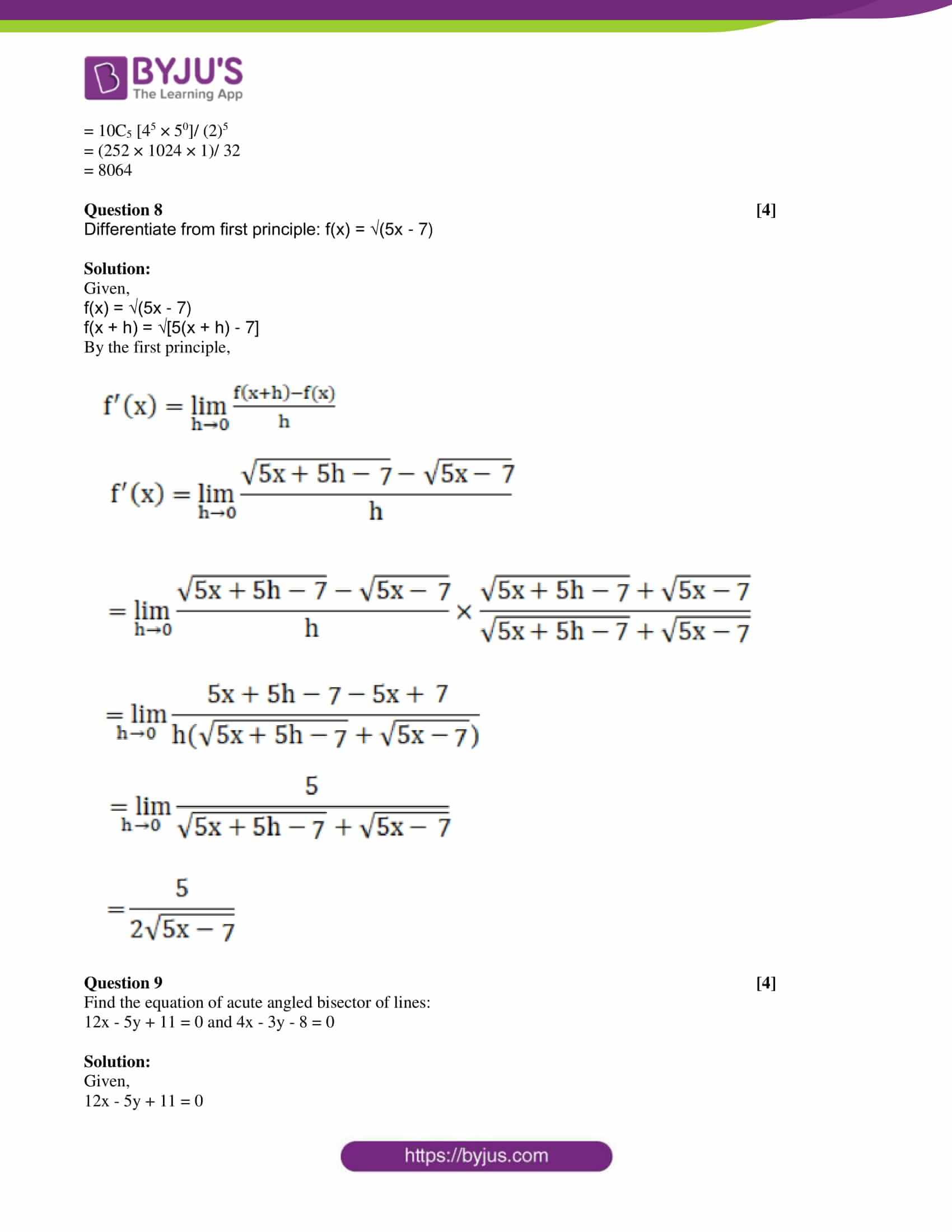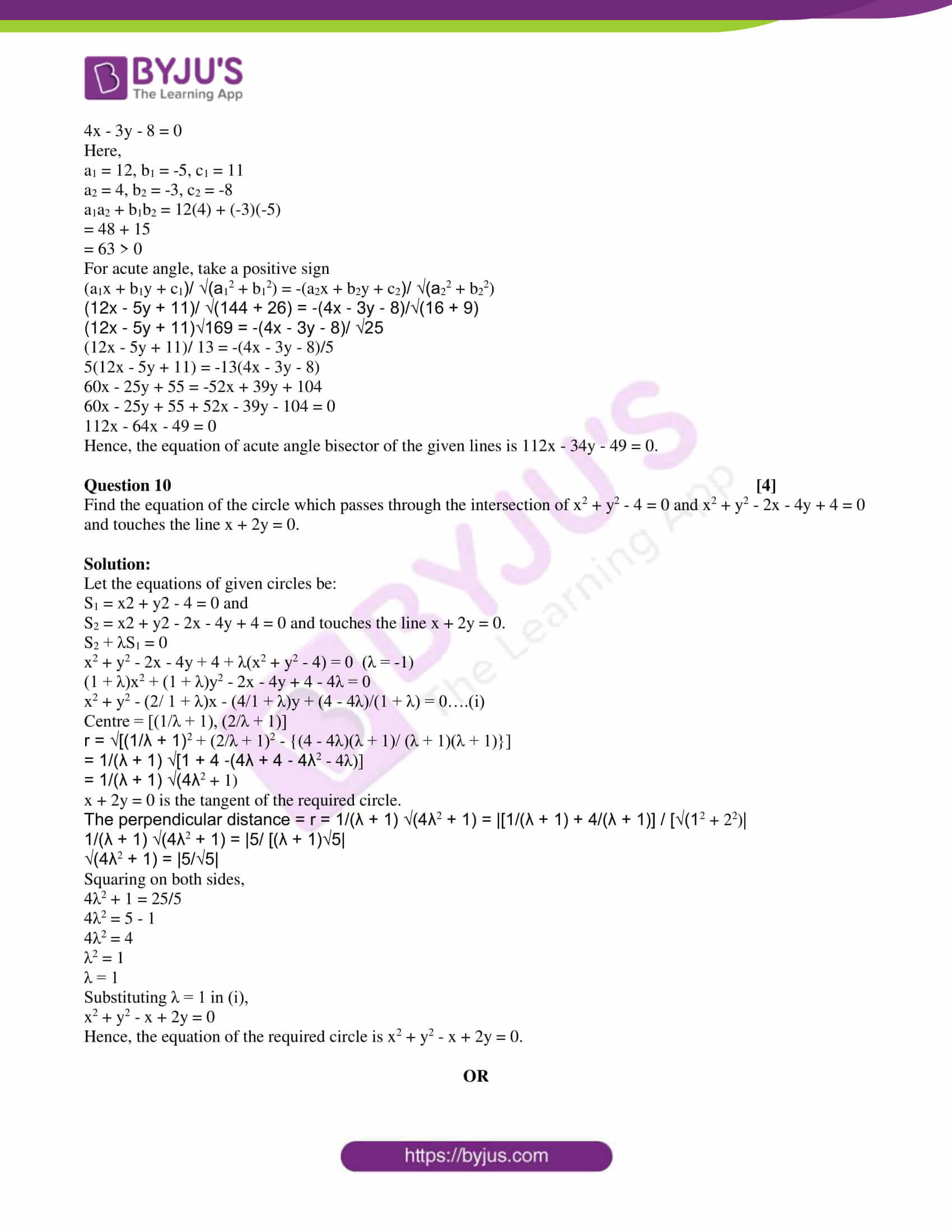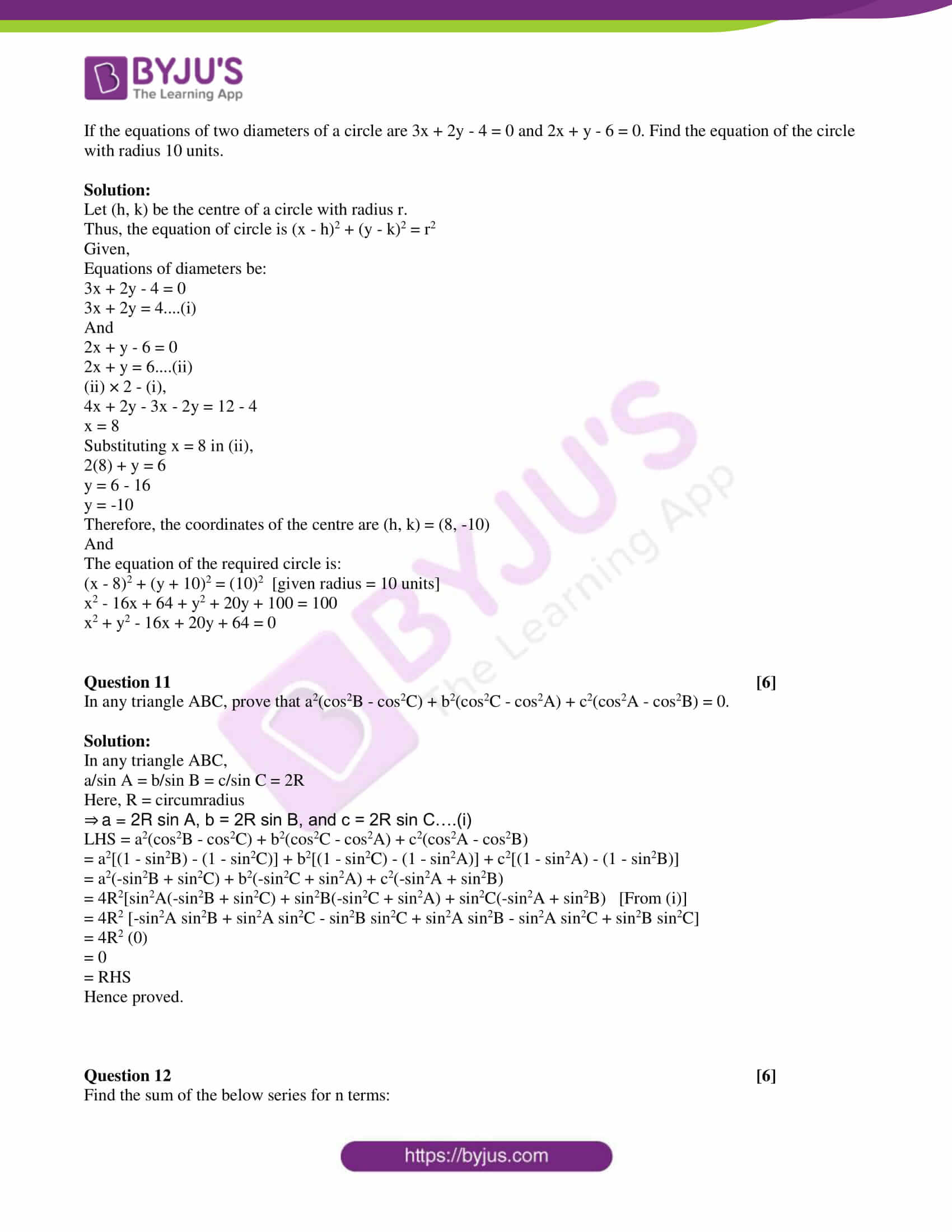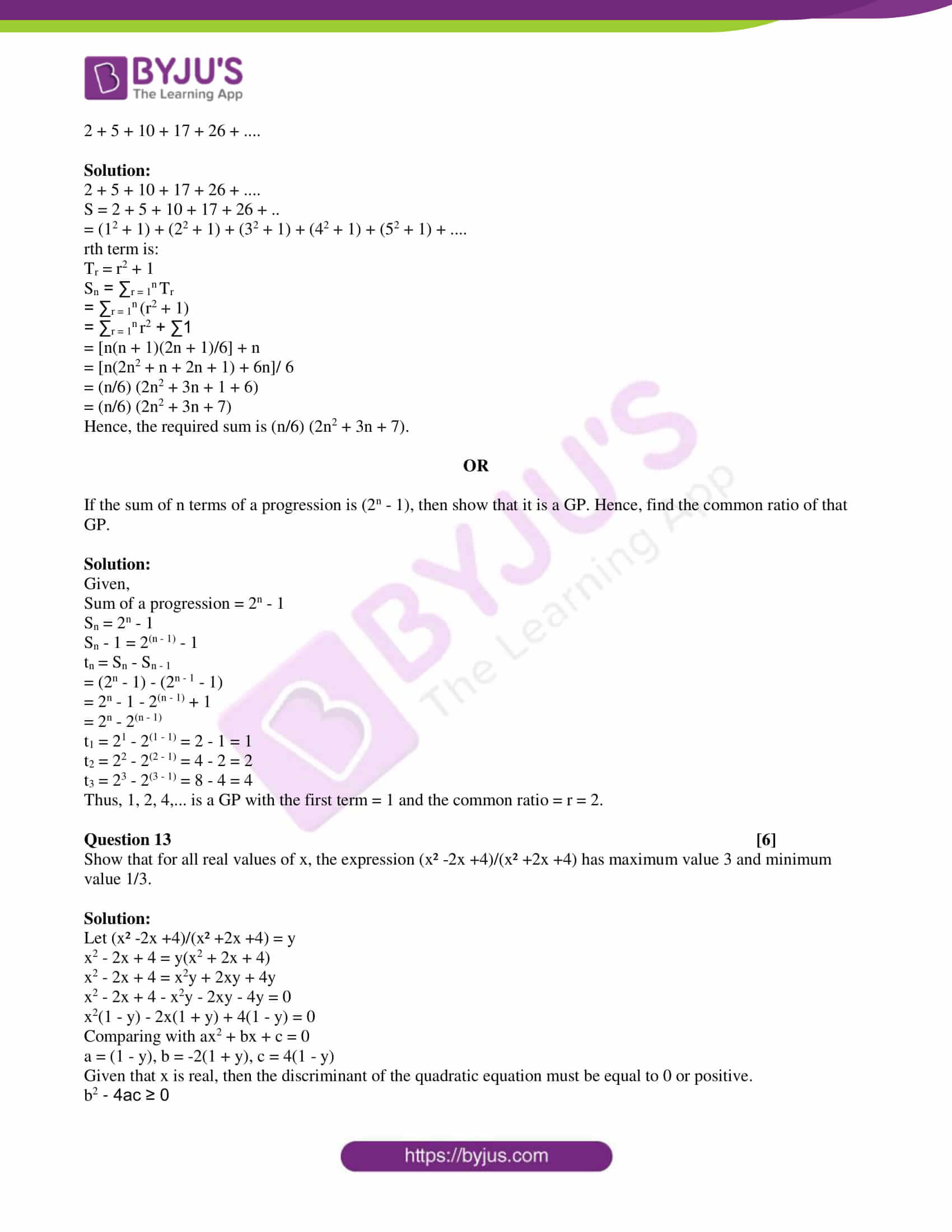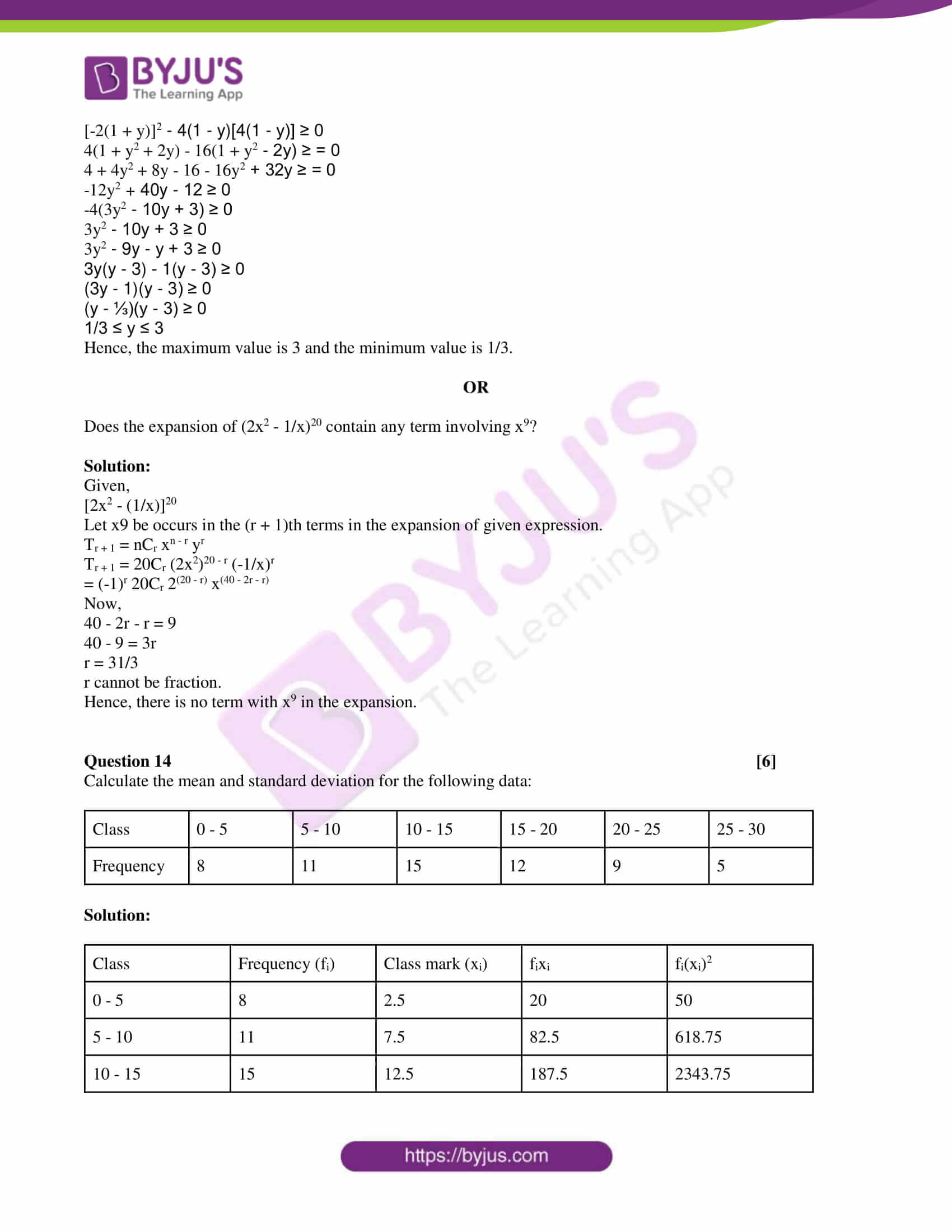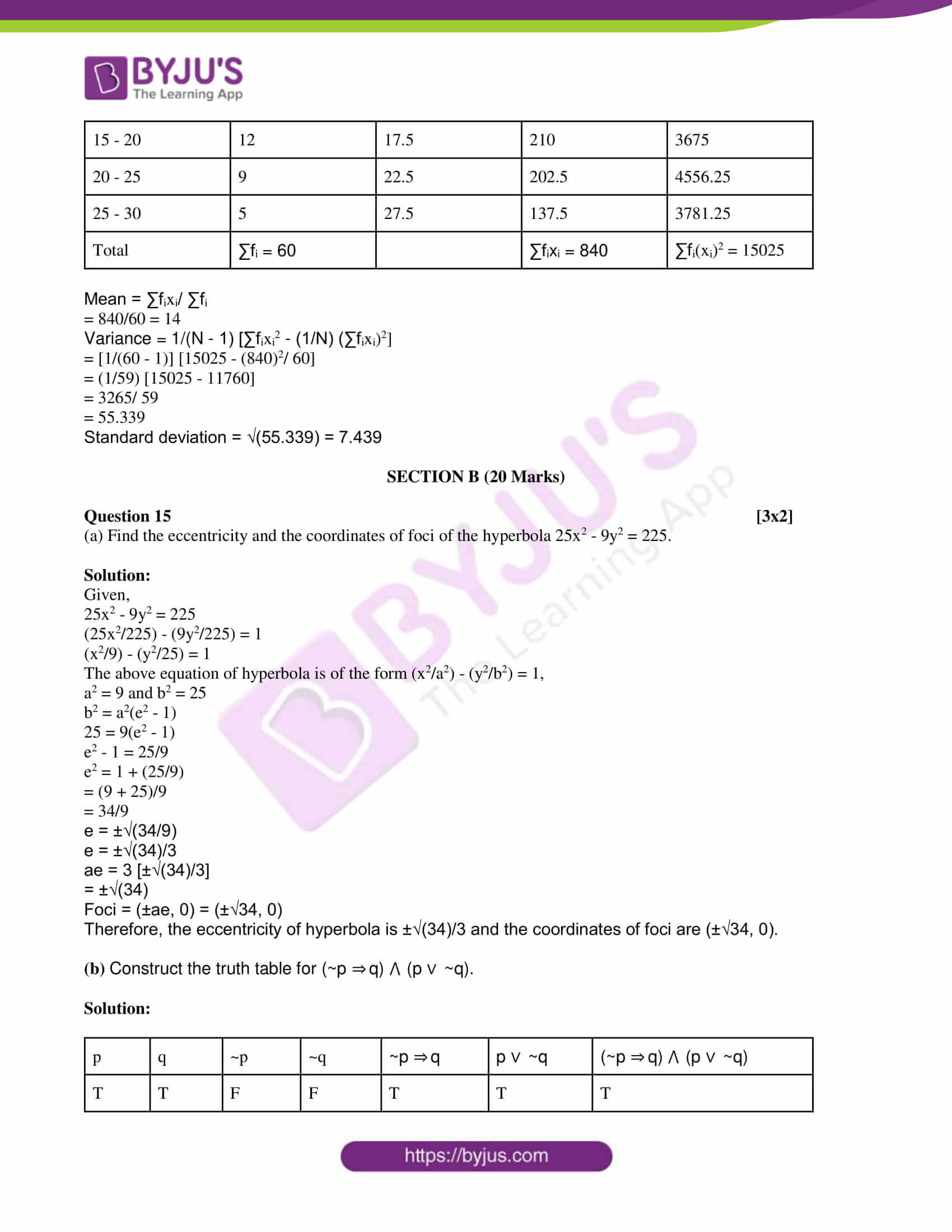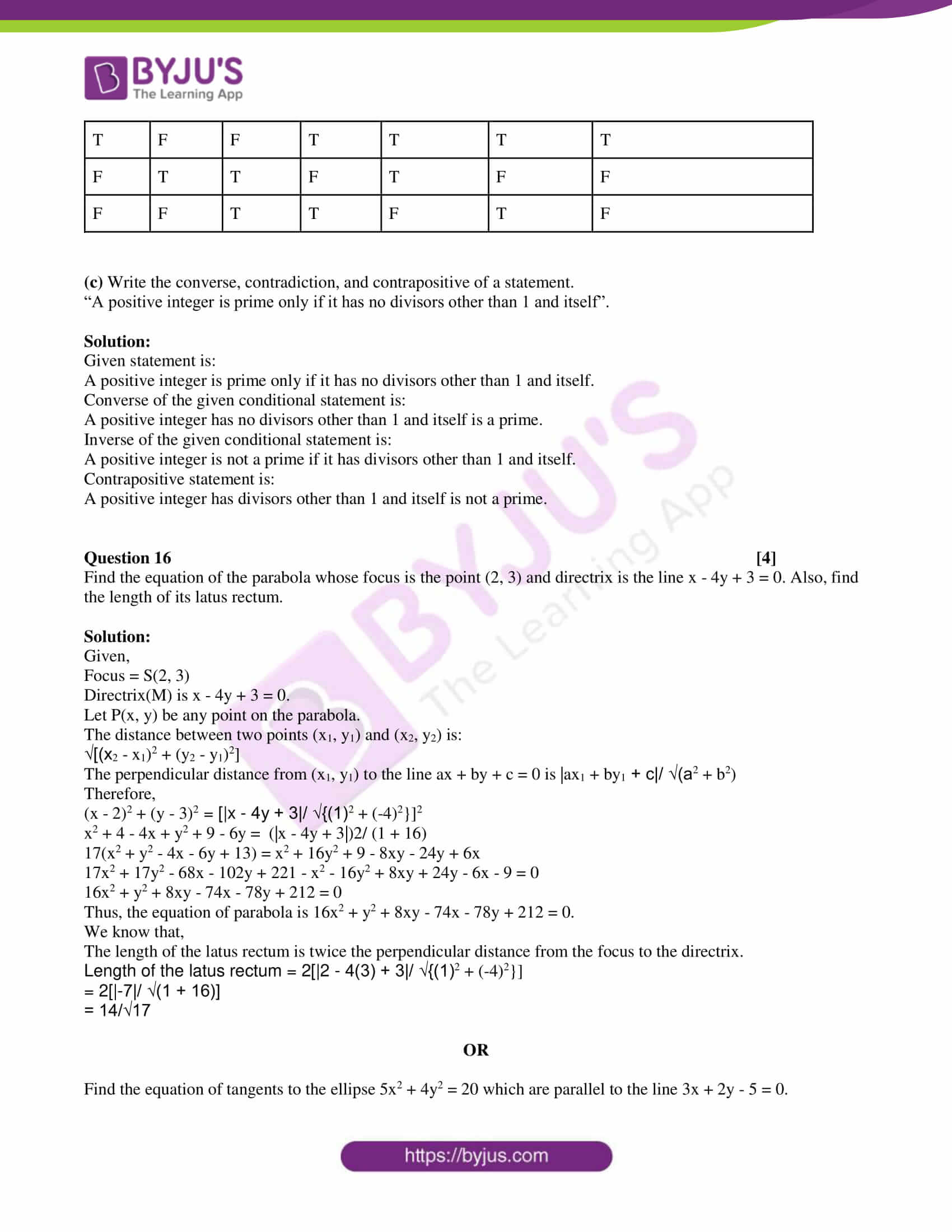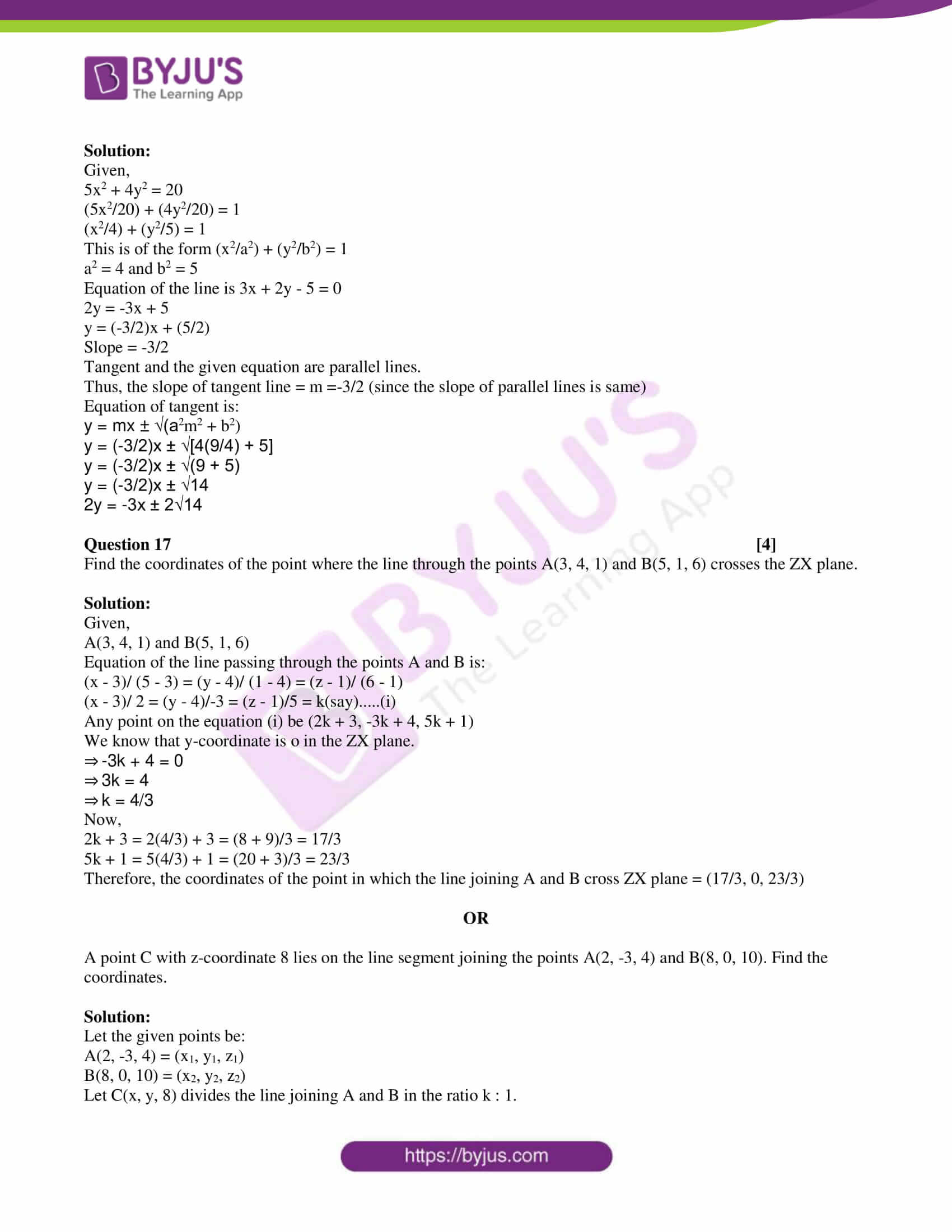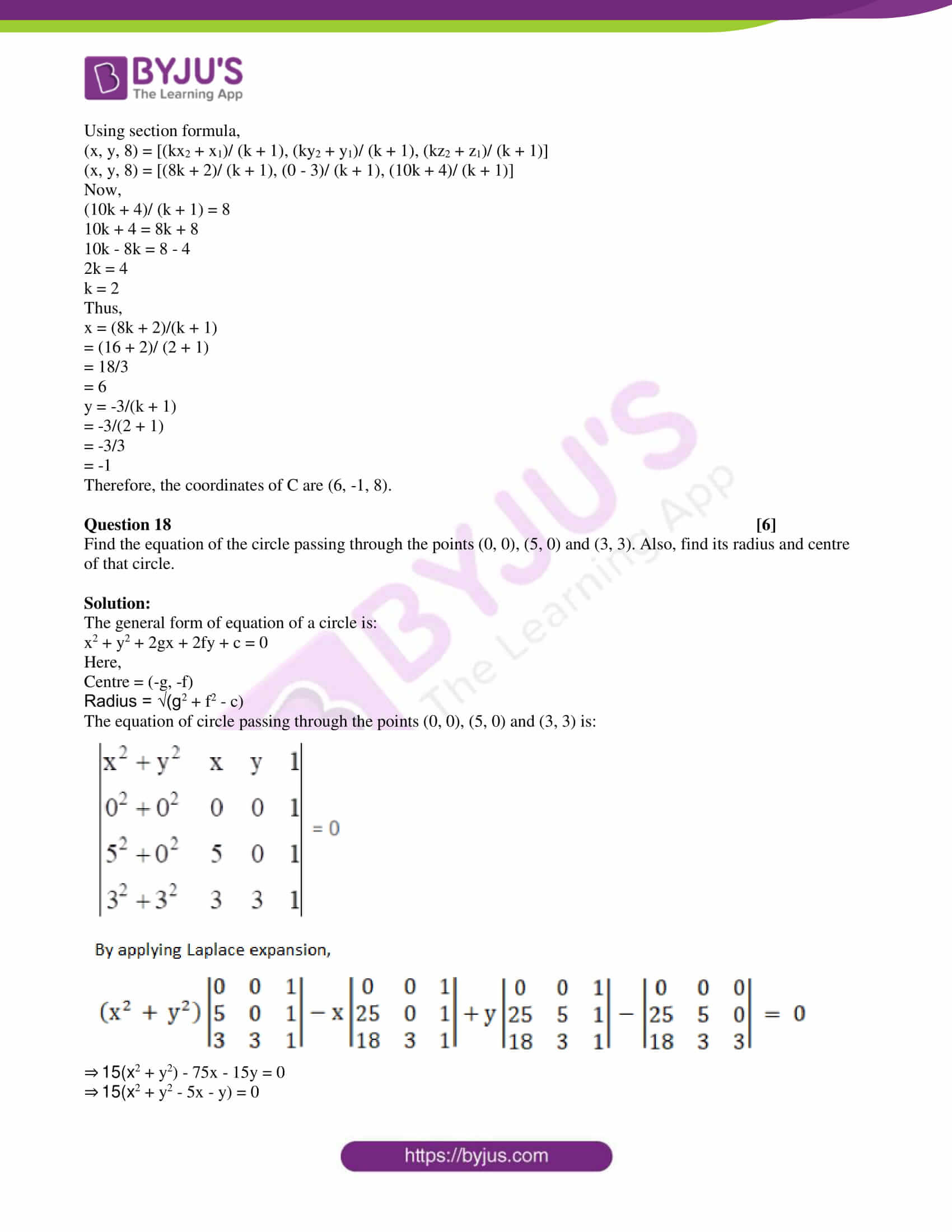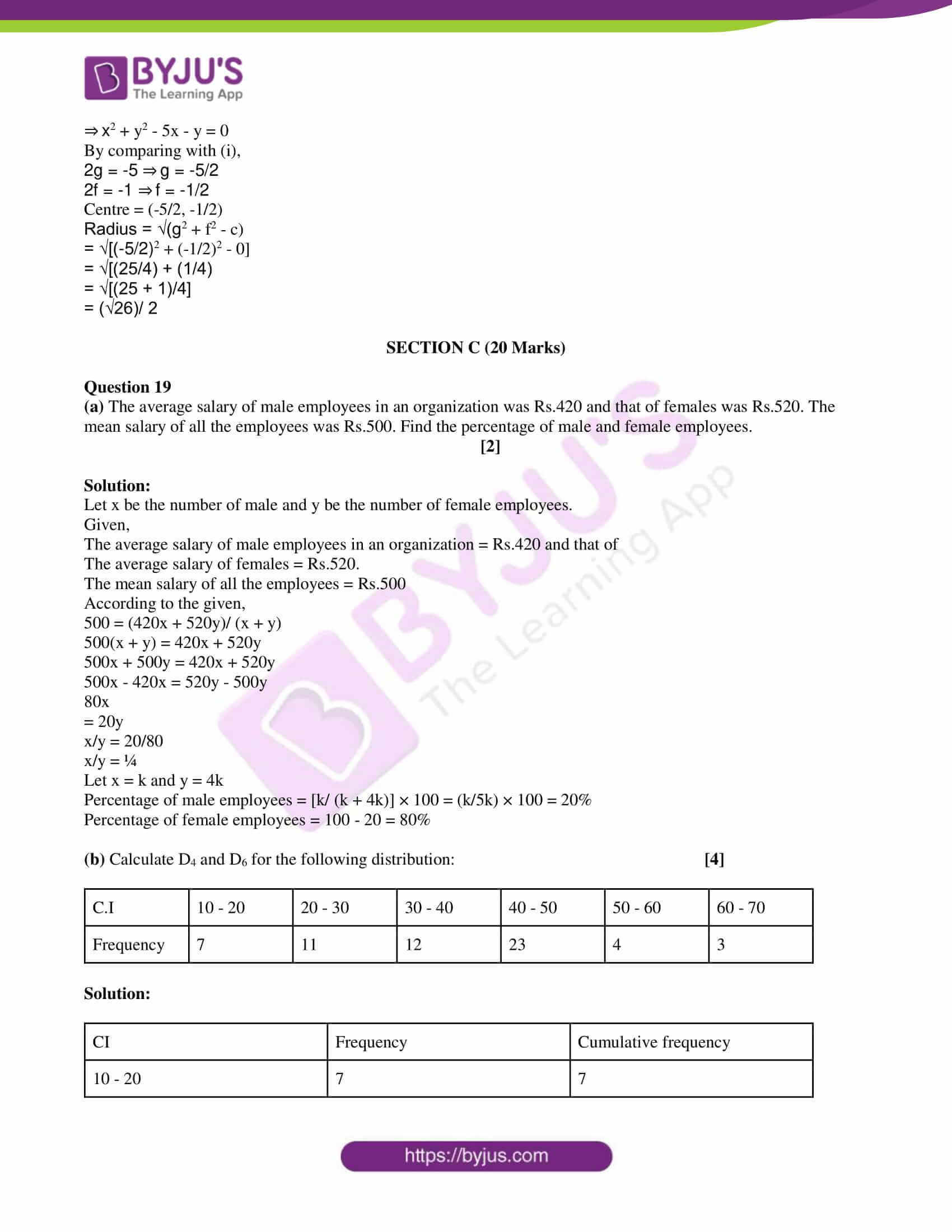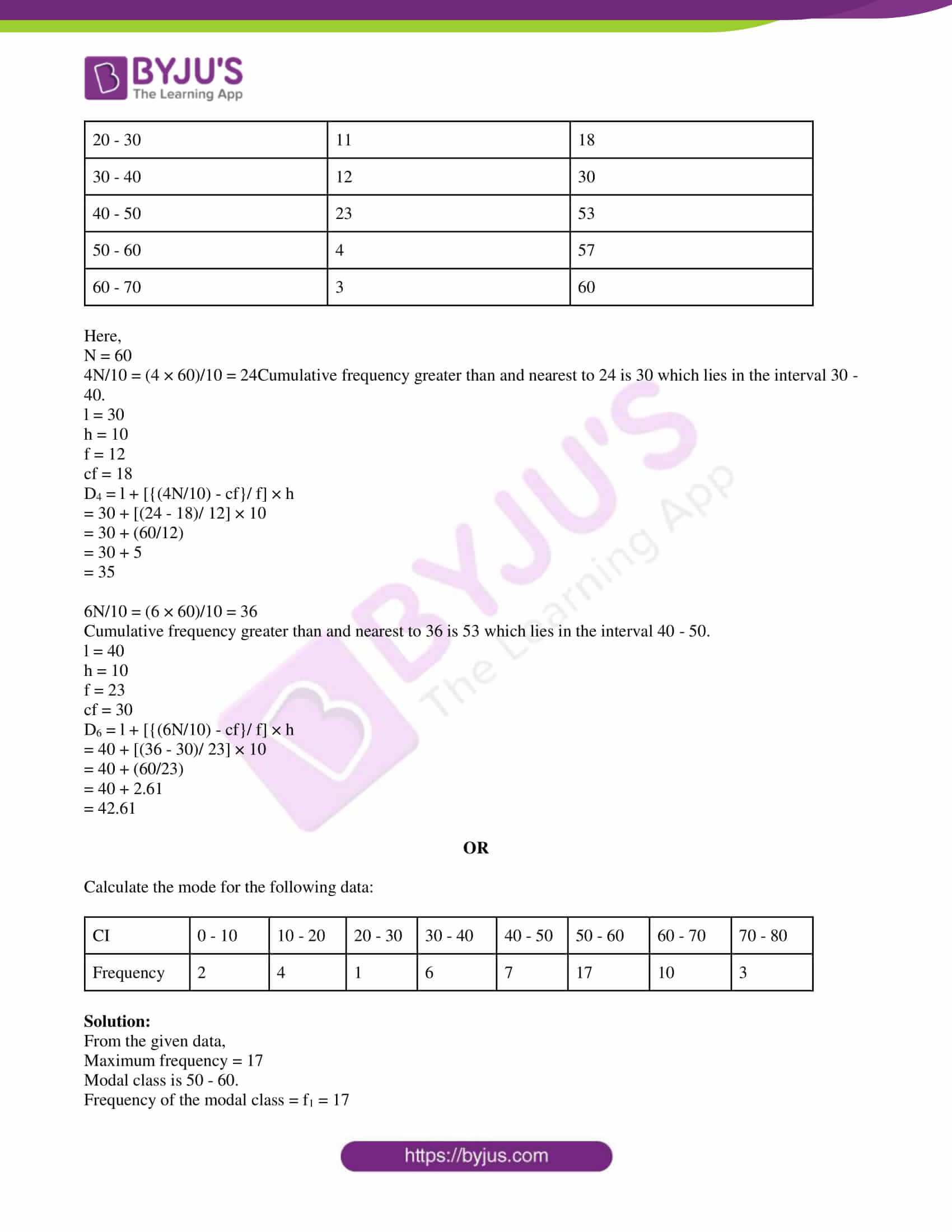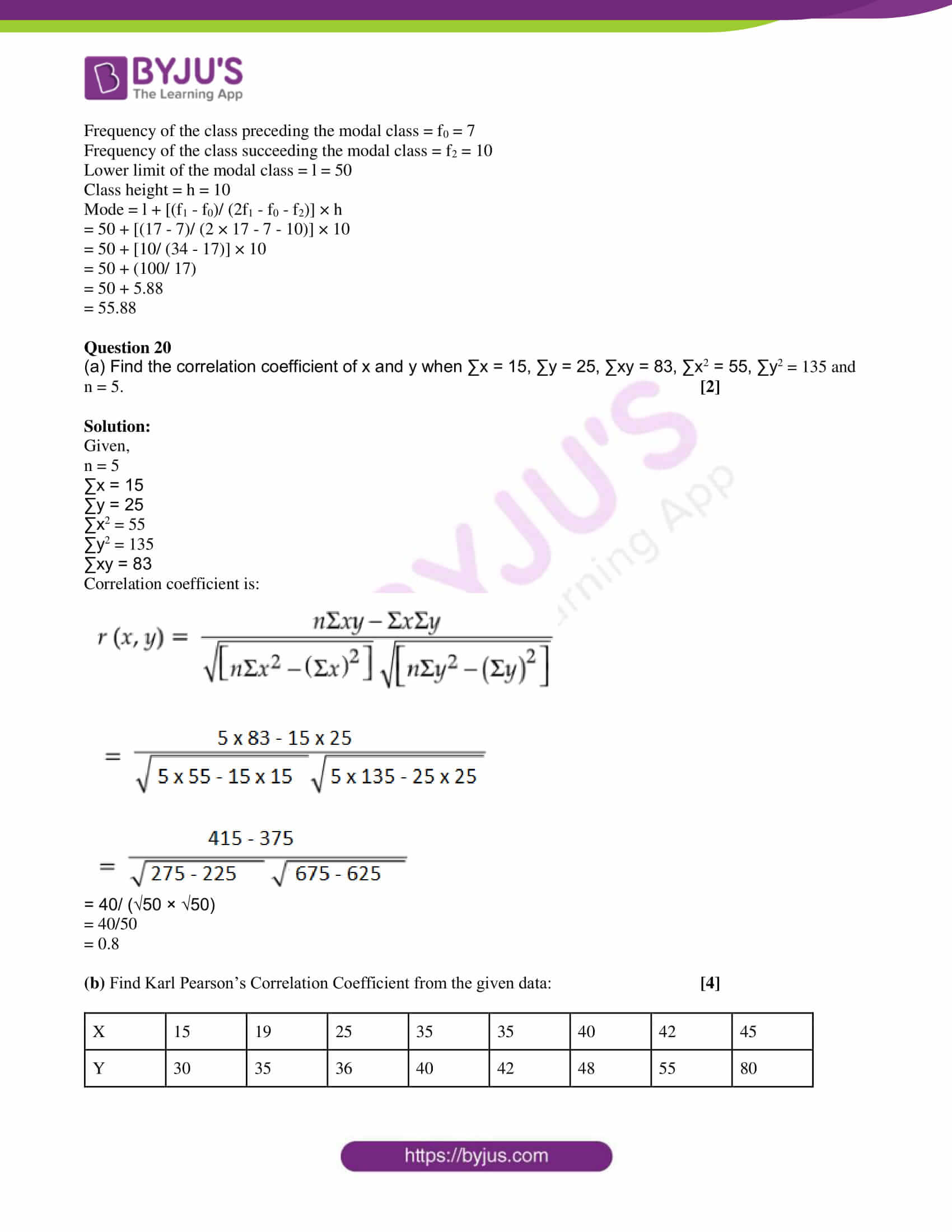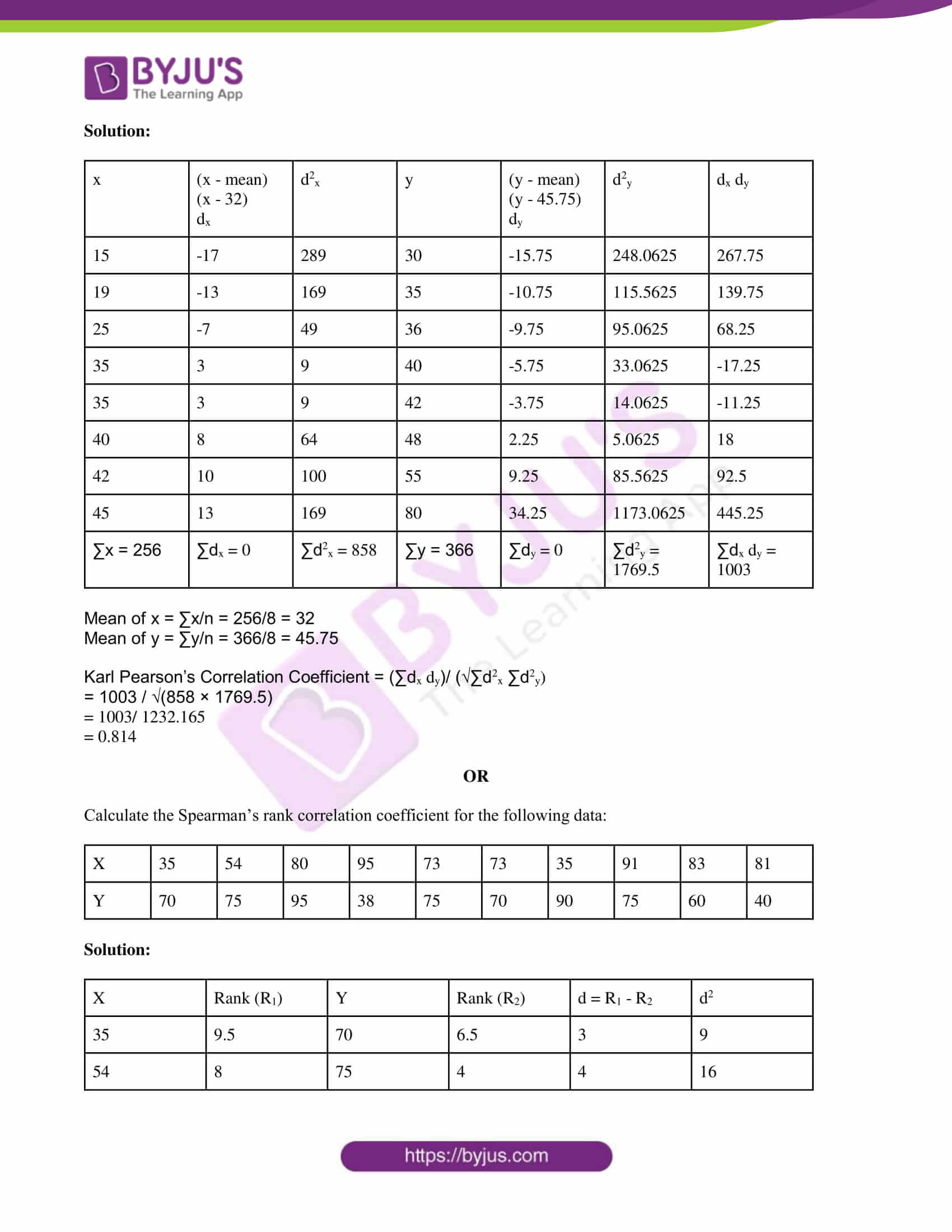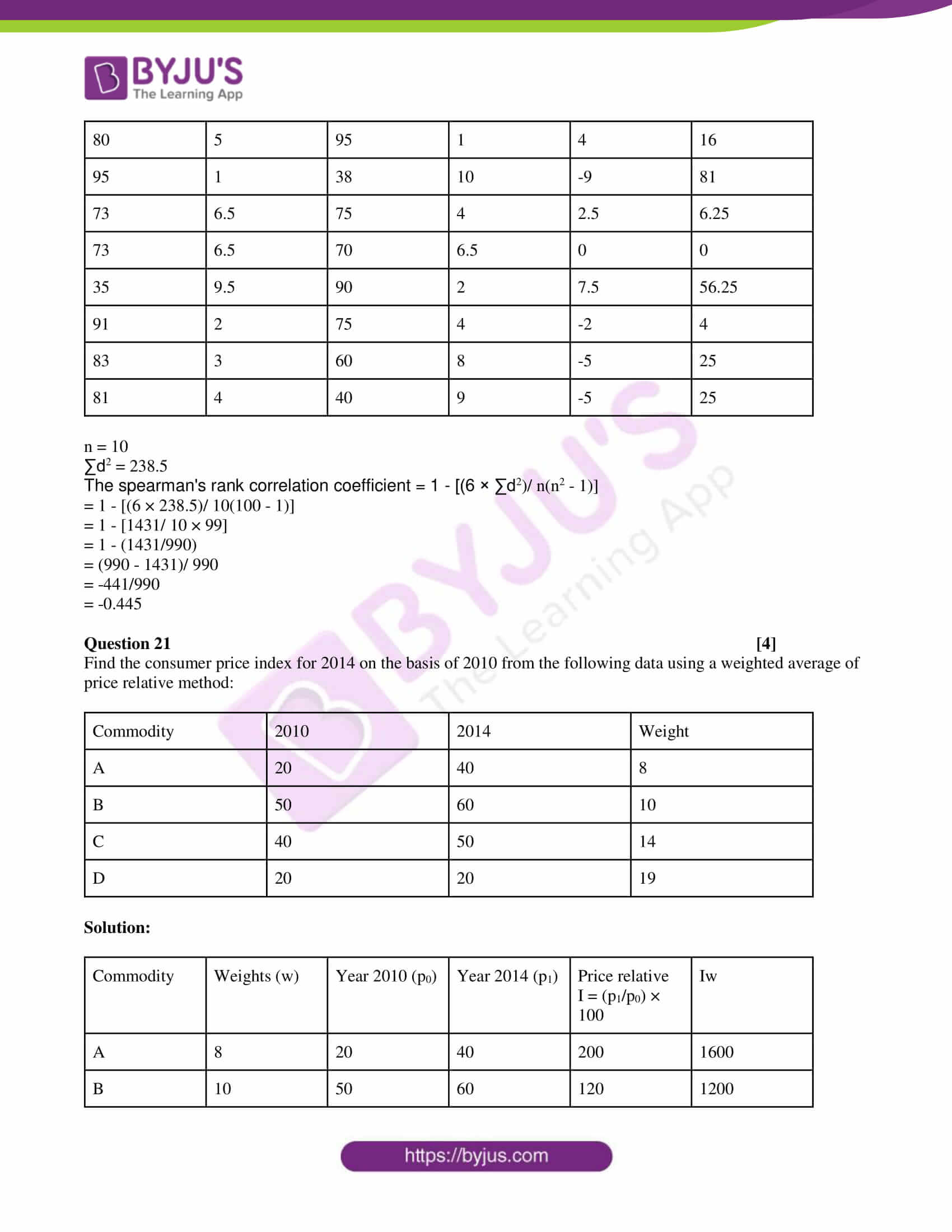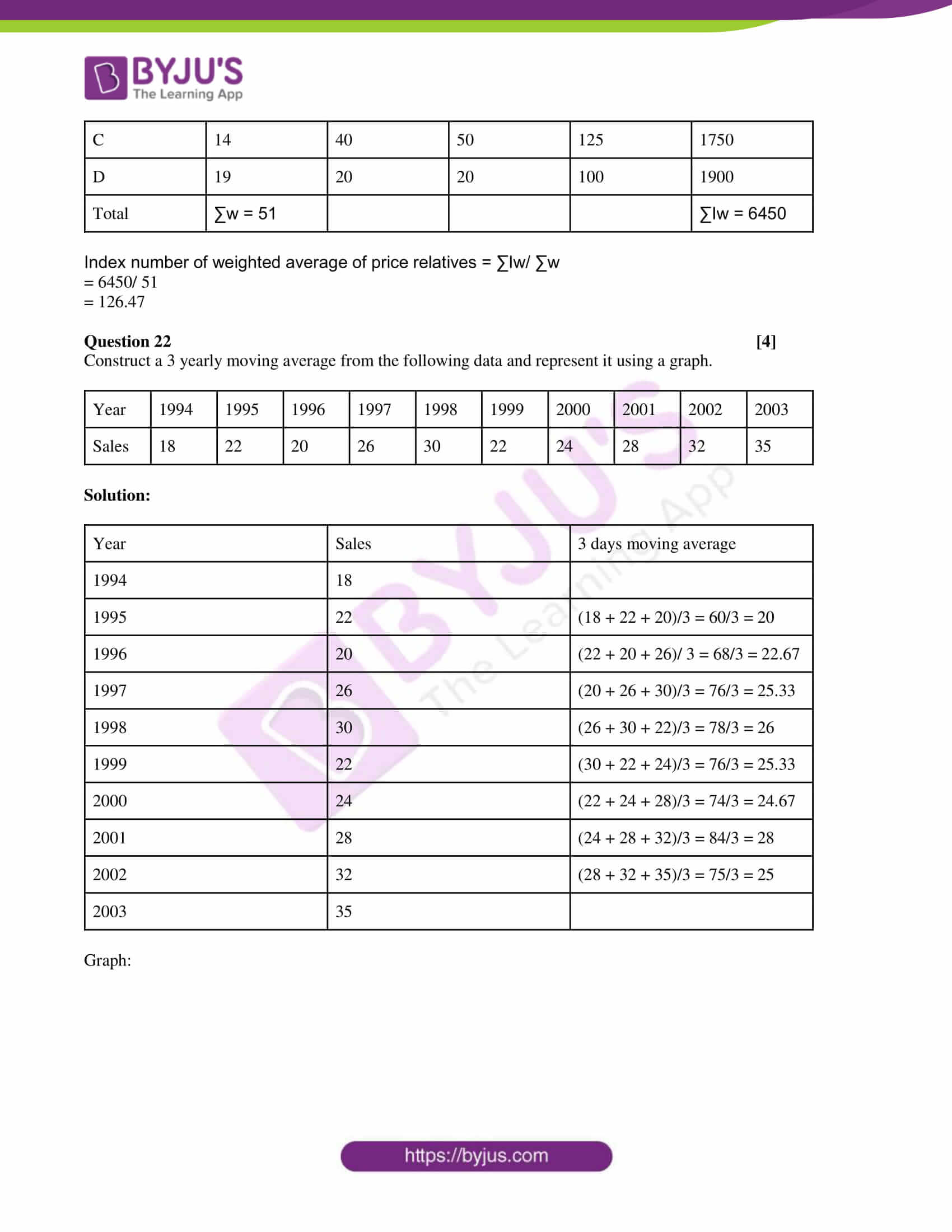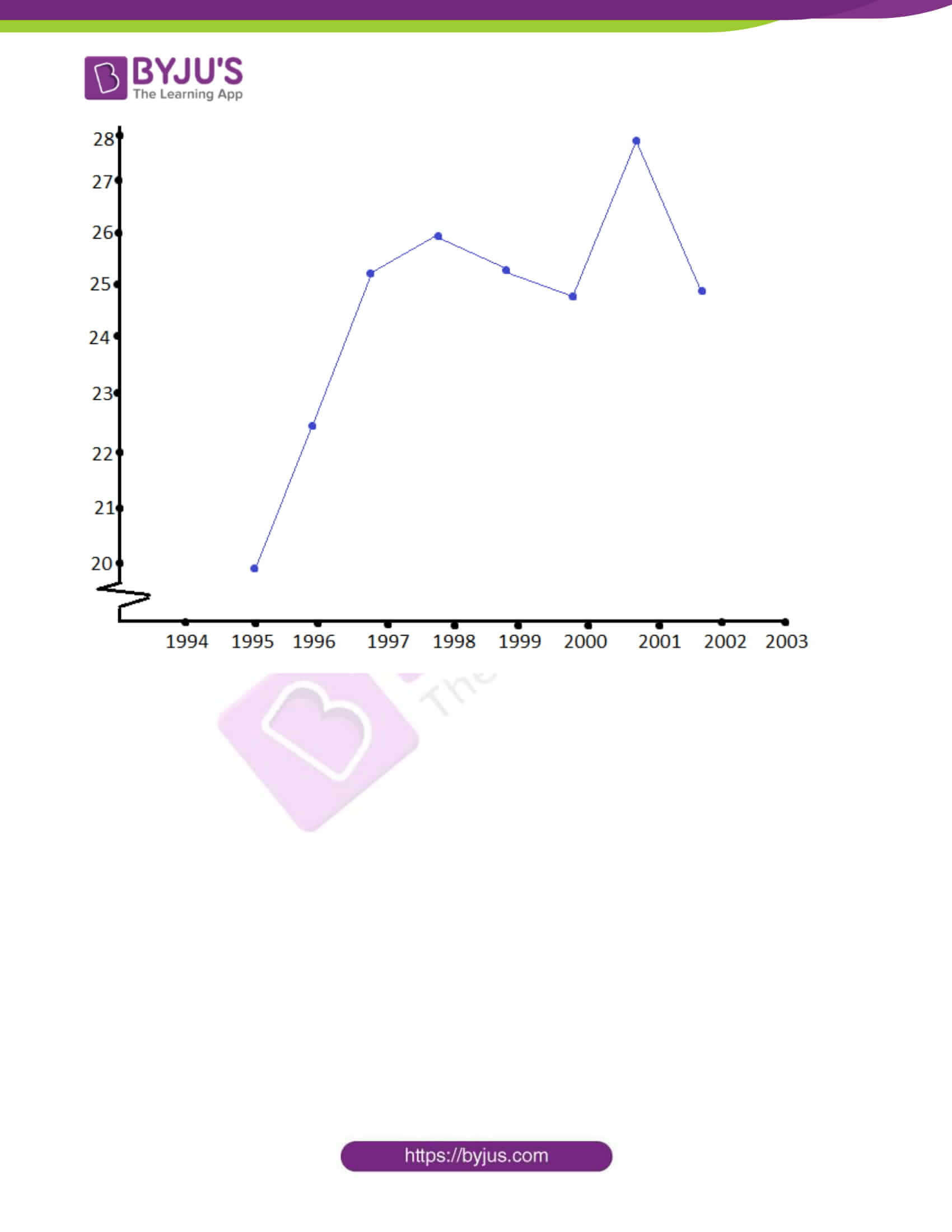SECTION – A

Question 1 [10×2]

(i) If U = {2, 3, 5, 7, 9} is the universal set and A = {3, 7}, B = {2, 5, 7, 9}, then prove that:

(a) (A ∪ B)’ = A’ ∩ B’

(b) (A ∩ B)’ = A’ ∪ B’

Solution:

Given,

U = {2, 3, 5, 7, 9}

A = {3, 7}

B = {2, 5, 7, 9}

(a) A ∪ B = {3, 7} ∪ {2, 5, 7, 9} = {2, 3, 5, 7, 9}

(A ∪ B)’ = U – (A ∪ B)

= {2, 3, 5, 7, 9} – {2, 3, 5, 7, 9}

= Φ

A’ = U – A

= {2, 3, 5, 7, 9} – {3, 7}

= {2, 5, 9}

B’ = U – B

= {2, 3, 5, 7, 9} – {2, 5, 7, 9}

= {3}

A’ ∩ B’ = {2, 5, 9} ∩ {3} = Φ

Therefore, (A ∪ B)’ = A’ ∩ B’

(b) A’ ∪ B’ = {2, 5, 9} ∪ {3} = {2, 3, 5, 9}

(A ∩ B) = {3, 7} ∩ {2, 5, 7, 9} = {7}

(A ∩ B)’ = U – (A ∩ B)

= {2, 3, 5, 7, 9} – {7}

= {2, 3, 5, 9}

Therefore, (A ∩ B)’ = A’ ∪ B’

(ii) If A = {x ∈ N : x ≤ 3} and B = {x ∈ W : x < 2}, find (A x B) and (B x A). Check whether (A x B) = (B x A)?

Solution:

Given,

A = {x ∈ N : x ≤ 3} and B = {x ∈ W : x < 2}

A = {1, 2, 3}

B = {0, 1}

A x B = {1, 2, 3} x {0, 1}

= {(1, 0), (1, 1), (2, 0), (2, 1), (3, 0), (3, 1)}

B x A = {0, 1} x {1, 2, 3}

= {(0, 1), (0, 2), (0, 3), (1, 1), (1, 2), (1, 3)}

(A x B) ≠ (B x A)

(iii) Find the domain and range of the function y = (x – 1)/ (x + 2).

Solution:

Given,

y = (x – 1)/ (x + 2)

Let f(x) = (x – 1)/ (x + 2)

f(x) is not defined for x = -2.

Thus, domain = = (-∞, -2) U (-2, ∞)

i.e. {x : x ∈ R and x ≠ -2}

y = (x – 1)/ (x + 2)

y(x + 2) = (x – 1)

xy + 2y = x – 1

2y + 1 = x – xy

2y + 1 = x(1 – y)

⇒ x = (2y + 1)/ (1 – y)

This is not defined for y = 1.

Therefore, y ≠ 1

Hence, the range of the given function = (-∞, 1) U (1, ∞)

i.e. {y : y ∈ R and y ≠ 1}

(iv)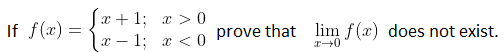Solution: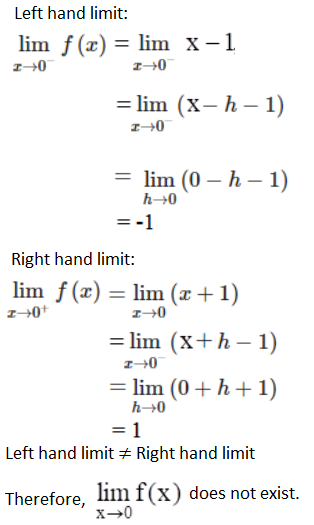(v) Using the principle of mathematical induction, prove the following for all n ∈ N.

2 + 6 + 18 + … + 2 × 3n – 1 = (3)n – 1

Solution:

Let P(n) = 2 + 6 + 18 + … + 2 × 3n – 1 = (3)n – 1

For n = 1,

LHS = 2

RJS = 31 – 1 = 3 – 1 = 2

P(n) is true for n = 1.

Let P(n) is true for n = k.

2 + 6 + 18 + … + 2 × 3k – 1 = (3)k – 1

Substituting n = k + 1

2 + 6 + 18 + … + 2 × 3k + 1 – 1 = (3)k + 1 – 1

2 + 6 + 18 + … + 2 × 3k – 1 + 2 × 3k = 3k + 1 – 1

Therefore, P(n) is also true for n = k + 1.

Hence proved.

(vi) Find the sum of the following series to infinity 8 + 4√2 + 4 + …∞

Solution:

Given,

8 + 4√2 + 4 + …∞

This is A GP with:

First term = a = 8

Common ratio = 4√2/ 8 = 4√2 / (4√2 × √2) = 1/√2

S = a/(1 – r)

= 8/ [1 – (1/√2)]

= 8/ [(√2 – 1)/ √2]

= 8√2/ (√2 – 1)

= [8√2/ (√2 – 1)] [(√2 + 1)/ (√2 + 1)]

= [8√2(√2 + 1)/ (2 – 1)

= 8(2) + 8√2

= 16 + 8√2

Therefore, the sum of the series to infinity is 16 + 8√2.

(vii) Solve the equation cos θ + cos 3θ – 2 cos 2θ = 0

Solution:

cos θ + cos 3θ – 2 cos 2θ = 0

2 cos [(θ + 3θ)/2] cos [(θ – 3θ)/2] – 2 cos 2θ = 0

2 cos 2θ cos (-θ) – 2 cos 2θ = 0

2 cos 2θ (cos θ – 1) = 0

2 cos 2θ = 0, cos θ – 1 = 0

cos 2θ = 0, cos θ = 1

2θ = 2nπ ± (π/2), θ = 2nπ ± 0

θ = nπ ± (π/4), θ = 2nπ

(viii) If two dice are thrown simultaneously, find the probability of getting a sum less than 7 or a doublet.

Solution:

Given,

Two dice are thrown simultaneously.

Total number of outcomes = 36

i.e. n(S) = 36

Let A be the event of getting a sum less than 7.

I.e. sum = 2, 3, 4, 5, 6

A = {(1, 1), (1, 2), (2, 1), (1, 3), (3, 1), (2, 2), (1, 4), (4, 1), (2, 3), (3, 2), (1, 5), (5, 1), (2, 4), (4, 2), (3, 3)}

n(A) = 15

P(A) = n(A)/n(S) = 15/36

Let B be the event of getting a doublet.

B = {(1, 1), (2, 2), (3, 3), (4, 4), (5, 5), (6, 6)}

n(B) = 6

P(B) = n(B)/n(S) = 6/36

P(A U B) = P(A) + P(B)

= (15/36) + (6/36)

= 21/36

= 7/12

Hence, the probability of getting a sum less than 7 or a doublet is 7/12.

(ix) Find the number of different signals that can be generated by arranging at least 2 flags in order (on below the other) on a vertical staff, if five different flags are available.

Solution:

Signals that can be generated by arranging at least 2 flags means a signal can consist of 2 or more i.e. either 2 flags, 3 flags, 4 flags or 5 flags.

Number of ways of generating signal with 2 different flags = 5P2

Number of ways of generating signal with 3 different flags = 5P3

Number of ways of generating signal with 4 different flags = 5P4

Number of ways of generating signal with 5 different flags = 5P5

Total number of ways = 5P2 + 5P3 + 5P4 + 5P5

= (5 × 4) + (5 × 4 × 3) + (5 × 4 × 3 × 2) + (5 × 4 × 3 × 2 × 1)

= 20 + 60 + 120 + 120

= 320

(x) Find the real values of x and y, if (x + iy)(2 – 3i) = 4 + i.

Solution:

Given,

(x + iy)(2 – 3i) = 4 + i

2x + i(-3x) + i(2y) – 3y(i)(i) = 4 + i

2x + i(-3x + 2y) – 3y(i2) = 4 + i

2x + i(-3x + 2y) – 3y(-1) = 4 + i

(2x + 3y) + i(-3x + 2y) = 4 + i

By comparing the corresponding terms,

2x + 3y = 4….(i)

-3x + 2y = 1….(ii)

(i) × 3 + (ii) × 2,

3(2x + 3y) + 2(-3x + 2y) = 3(4) + 2(1)

6x + 9y – 6x + 4y = 12 + 2

13y = 14

y = 14/13

Substituting y = 14/13 in (i),

2x + 3(14/13) = 4

2x = 4 – (42/13)

2x = (52 – 42)/13

2x = 10/13

x = 5/13

Therefore, x = 5/13 and y = 14/13

Question 2 

Draw the graph of the function y = |x – 4| + |x – 7|.

Solution:

|x – 4| = x – 4 if x > 4, and |x – 4| = -x + 4 if x < 4

Similarly,

|x – 7| = x – 7 if x > 7, and |x – 7| = -x + 7 if x < 7.

If x < 4, then |x – 4| + |x – 7| = -2x + 11.

If x > 4, then |x – 4| + |x – 7| = 2x – 11.

Now consider another case:

When x is between the values of 4 and 7 then |x – 4| = x – 4 and |x – 7| = -x + 7.

⇒ |x – 4| + |x – 7| = (x – 4) + (-x + 7) = 3

Thus, for x values between 4 and 7, we get the equation y = 3, which is just a horizontal line.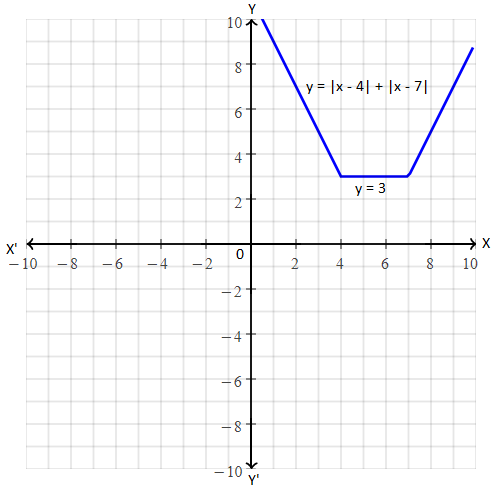Question 3 

Solve the following equation:

tan x + tan 2x + tan 3x = 0

Solution:

tan x + tan 2x + tan 3x = 0

tan x + tan 2x = -tan 3x

(sin x/cos x) + (sin 2x/cos 2x) = -sin 3x/cos 3x

(sin x cos 2x + cos x sin 2x)/ (cos x cos 2x) = -sin 3x/cos 3x

sin (2x + x) cos 3x = – cos x cos 2x sin 3x

sin 3x cos 3x + cos x cos 2x sin 3x = 0

sin 3x (cos 3x + cos x cos 2x) = 0

sin 3x [cos (2x + x ) + cos x cos 2x] = 0

sin 3x [ cos x cos 2x – sin x sin 2x + cos x cos 2x) = 0

– sin 3x sin x sin 2x = 0

sin x sin 2x sin 3x = 0

sin x = 0

⇒ x = nπ, n ∈ I

sin 2x = 0

⇒ 2x = nπ

⇒ x = (nπ/2), n ∈ I

sin 3x = 0

⇒ 3x = nπ

⇒ x = (nπ/3), n ∈ I

x = nπ/2 does not satisfy the given equation.

Hence, the required solution is x = nπ and x = nπ/3.

OR

In a triangle, ABC, prove that sin A + sin B + sin C = 4 cos A/2 cos B/2 cos C/2

Solution:

In any triangle ABC,

A + B + C = π

A + B = π – C

(A + B)/2 = (π – C)/2

(A + B)/2 = (π/2) – (C/2)

LHS = sin A + sin B + sin C

= 2 sin [(A + B)/2] cos [(A – B)/2] + sin C

= 2 sin [(π/2) – (C/2)] cos [(A – B)/2] + 2 sin (C/2) cos (C/2)

= 2 cos (C/2) cos [(A – B)/2] + 2 sin (C/2) cos (C/2)

= 2 cos (C/2) {cos [(A – B)/2] + sin (C/2)}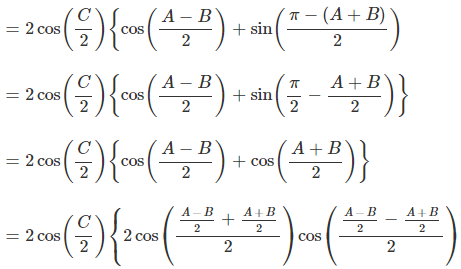= 2 cos (C/2) [2 cos (A/2) cos (-B/2)]

= 4 cos (A/2) cos (B/2) cos (C/2)

= RHS

Hence proved.

Question 4 

Prove that: 52n – 1 is divisible by 24 for all n ∈ N.

Solution:

Let P(n) = 52n – 1

For n = 1,

P(1) = 52(1) – 1 = 52 – 1 = 25 – 1 = 24

For n = 1, P(n) is divisible by 24.

Let P(n) be divisible by 24.

i.e. 52k – 1 = 24m, m ∈ N….(i)

For n = k + 1

P(k + 1) = 52(k + 1) – 1

= 5(2k + 2) – 1

= 52k 52 – 1

= 25(24m + 1) – 1 [From (i)]

= 600m + 24

= 24(25m + 1)

Which is divisible by 24.

P(n) is divisible for n = k + 1 also.

Hence proved.

Question 5 

Find the locus of the point z satisfying |z| + |z – i| = 3 where z = x + iy and represent it in the argand diagram.

Solution:

Given,

z = x + iy

|z| + |z – i| = 3

|x + iy| + |x + iy – i| = 3

|x + iy| + |x + i(y – 1)| = 3

√(x2 + y2) + √[x2 + (y – 1)2] = 3

√(x2 + y2) = 3 – √[x2 + (y – 1)2]

Squaring on both sides,

x2 + y2 = (3)2 + [x2 + (y – 1)2] – 6√[x2 + (y – 1)2]

x2 + y2 = 9 + x2 + y2 + 1 – 2y – 6√[x2 + (y – 1)2]

6√[x2 + (y – 1)2] = 9 – 2y

Again squaring on both sides,

36[x2 + (y – 1)2] = 81 + 4y2 – 36y

36x2 + 36(y2 + 1 – 2y) – 81 – 4y2 + 36y = 0

36x2 + 36y2 + 36 – 72y – 81 – 4y2 + 36y = 0

36x2 + 32y2 – 36y – 45 = 0

36x2 + 32[y2 – (9y)/8] = 45

36x2 + 32 [y2 – 2(9y/16) + (9/16)2] = 45 + 32(9/16)2

36x2 + 32 [y – (9/16)]2 = 45 + (81/8)

36x2 + 32[y – (9/16)]2 = (441/8)

36x2 + 32[y – (9/16)]2 = (21/ 2√2)2

⇒ [(x – 0)2/ (7/4√2)2] + [{y – (9/16}2/ (21/16)2] = 1

Thus, the locus of z represents an ellipse with centre (0, 9/16).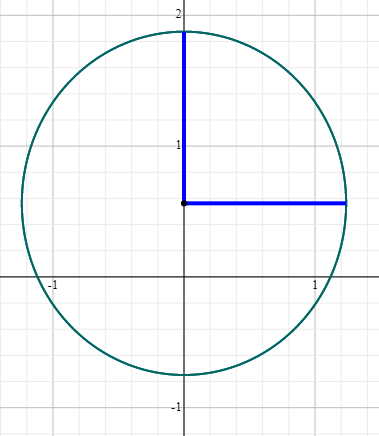Question 6 

A committee of 5 persons has to be chosen from 8 boys and 6 girls consisting of:

(i) at least 4 girls

(ii) more boys than girls.

Solution:

Given,

Number of boys = 8

Number of girls = 6

The number of members should be in the committee = 5

(i) At least 4 girls

The committee consists of 4 girls + 1 boys and 5 girls + 0 boys

Total number of ways in this case = 6C4 × 8C1 + 6C5 × 8C0

= 6C2 × 8 + 6C1 × 1

= [(6 × 5)/ (2 × 1)] × 8 + 6

= 120 + 6

= 126

(iii) More boys than girls

(No girl + 5 boys) or (1 girl + 4 boys) or (2 girls + 3 boys)

No girl + 5 boys = 6C0 × 8C5 = 1 × 8C3 = (8 × 7 × 6)/ (3 × 2 × 1) = 56

1 girls + 4 boys = 6C1 × 8C4 = 6 × [(8 × 7 × 6 × 5)/ (4 × 3 × 2 × 1)] = 70

2 girls + 3 boys = 6C2 × 8C3 = [(6 × 5)/ (2 × 1)] × [(8 × 7 × 6)/ (3 × 2 × 1)] = 15 × 56 = 840

Total number of ways = 56 + 70 + 840 = 966

OR

How many words each of 3 vowels and 2 consonants can be formed from the letters of the word INVOLUTE?

Solution:

Given word is:

INVOLUTE

Total number of letters = 8

Total vowels = {I, O, U, E} = 4

Total consonants = {N, V, L, T} = 4

Number of ways to select 3 vowels is 4C3.

And the number of ways to select 2 consonants is 4C2.

Thus, the number of ways to arrange these 5 letters = 4C3 × 4C2 × 5!

Using the formula,

nCr = n!/r!(n – r)!

4C3 = 4!/3!(4 – 3)!

= 4!/(3! 1!)

= [4 × 3!] / 3!

= 4

4C2 = 4!/2!(4 – 2)!

= 4!/(2! 2!)

= [4 × 3 × 2!] / (2! 2!)

= (4 × 3) / (2 × 1)

= 2 × 3

= 6

By substituting these values,

4C3 × 4C2 × 5! = 4 × 6 × 5!

= 4 × 6 × (5 × 4 × 3 × 2 × 1)

= 2880

Hence, the number of words that can be formed containing 3 vowels and 2 consonants chosen from ‘INVOLUTE’ is 2880.

Question 7 

Find the term independent of x in the expansion of [(4x/5) + (5/2x)]10.

Solution:

[(4x/5) + (5/2x)]10

We know that the general term of (a + b)n is:

Tr + 1 = nCr (a)n – r (a)r

Tr + 1 = 10Cr (4x/5)10 – r . (5/ 2x)r

= 10Cr 4(10 – r)(x/5)(10 – r) . (5)r [2-r x-r]

= 10Cr (4) (10 – r) (5)-(10 – r) + r 2-r x[(10 – r) – r]

To get the term which is independent of x, assume the value of power of x as 0.

[(10 – r) – r] = 0

10 – 2r = 0

2r = 10

r = 5

Thus, the fifth term will be the required term.

T5 + 1 = 10C5 (4) (10 – 5) (5)2(5) – 10 2-5

= 10C5 [45 × 50]/ (2)5

= (252 × 1024 × 1)/ 32

= 8064

Question 8 

Differentiate from first principle: f(x) = √(5x – 7)

Solution:

Given,

f(x) = √(5x – 7)

f(x + h) = √[5(x + h) – 7]

By the first principle,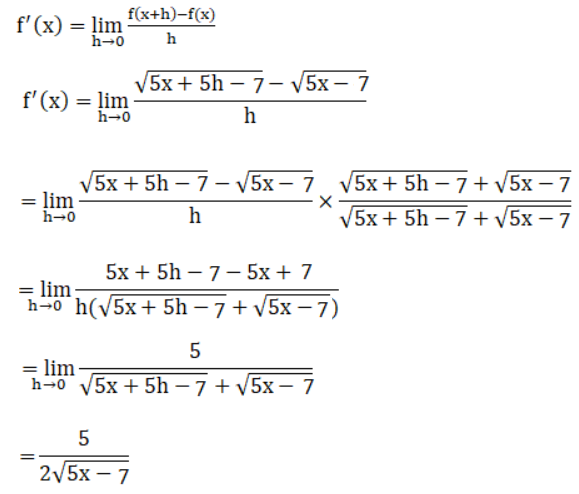Question 9 

Find the equation of acute angled bisector of lines:

12x – 5y + 11 = 0 and 4x – 3y – 8 = 0

Solution:

Given,

12x – 5y + 11 = 0

4x – 3y – 8 = 0

Here,

a1 = 12, b1 = -5, c1 = 11

a2 = 4, b2 = -3, c2 = -8

a1a2 + b1b2 = 12(4) + (-3)(-5)

= 48 + 15

= 63 > 0

For acute angle, take a positive sign

(a1x + b1y + c1)/ √(a12 + b12) = -(a2x + b2y + c2)/ √(a22 + b22)

(12x – 5y + 11)/ √(144 + 26) = -(4x – 3y – 8)/√(16 + 9)

(12x – 5y + 11)√169 = -(4x – 3y – 8)/ √25

(12x – 5y + 11)/ 13 = -(4x – 3y – 8)/5

5(12x – 5y + 11) = -13(4x – 3y – 8)

60x – 25y + 55 = -52x + 39y + 104

60x – 25y + 55 + 52x – 39y – 104 = 0

112x – 64x – 49 = 0

Hence, the equation of acute angle bisector of the given lines is 112x – 34y – 49 = 0.

Question 10 

Find the equation of the circle which passes through the intersection of x2 + y2 – 4 = 0 and x2 + y2 – 2x – 4y + 4 = 0 and touches the line x + 2y = 0.

Solution:

Let the equations of given circles be:

S1 = x2 + y2 – 4 = 0 and

S2 = x2 + y2 – 2x – 4y + 4 = 0 and touches the line x + 2y = 0.

S2 + λS1 = 0

x2 + y2 – 2x – 4y + 4 + λ(x2 + y2 – 4) = 0 (λ = -1)

(1 + λ)x2 + (1 + λ)y2 – 2x – 4y + 4 – 4λ = 0

x2 + y2 – (2/ 1 + λ)x – (4/1 + λ)y + (4 – 4λ)/(1 + λ) = 0….(i)

Centre = [(1/λ + 1), (2/λ + 1)]

r = √[(1/λ + 1)2 + (2/λ + 1)2 – {(4 – 4λ)(λ + 1)/ (λ + 1)(λ + 1)}]

= 1/(λ + 1) √[1 + 4 -(4λ + 4 – 4λ2 – 4λ)]

= 1/(λ + 1) √(4λ2 + 1)

x + 2y = 0 is the tangent of the required circle.

The perpendicular distance = r = 1/(λ + 1) √(4λ2 + 1) = |[1/(λ + 1) + 4/(λ + 1)] / [√(12 + 22)|

1/(λ + 1) √(4λ2 + 1) = |5/ [(λ + 1)√5|

√(4λ2 + 1) = |5/√5|

Squaring on both sides,

2 + 1 = 25/5

2 = 5 – 1

2 = 4

λ2 = 1

λ = 1

Substituting λ = 1 in (i),

x2 + y2 – x + 2y = 0

Hence, the equation of the required circle is x2 + y2 – x + 2y = 0.

OR

If the equations of two diameters of a circle are 3x + 2y – 4 = 0 and 2x + y – 6 = 0. Find the equation of the circle with radius 10 units.

Solution:

Let (h, k) be the centre of a circle with radius r.

Thus, the equation of circle is (x – h)2 + (y – k)2 = r2

Given,

Equations of diameters be:

3x + 2y – 4 = 0

3x + 2y = 4….(i)

And

2x + y – 6 = 0

2x + y = 6….(ii)

(ii) × 2 – (i),

4x + 2y – 3x – 2y = 12 – 4

x = 8

Substituting x = 8 in (ii),

2(8) + y = 6

y = 6 – 16

y = -10

Therefore, the coordinates of the centre are (h, k) = (8, -10)

And

The equation of the required circle is:

(x – 8)2 + (y + 10)2 = (10)2 [given radius = 10 units]

x2 – 16x + 64 + y2 + 20y + 100 = 100

x2 + y2 – 16x + 20y + 64 = 0

Question 11 

In any triangle ABC, prove that a2(cos2B – cos2C) + b2(cos2C – cos2A) + c2(cos2A – cos2B) = 0.

Solution:

In any triangle ABC,

a/sin A = b/sin B = c/sin C = 2R

⇒ a = 2R sin A, b = 2R sin B, and c = 2R sin C….(i)

LHS = a2(cos2B – cos2C) + b2(cos2C – cos2A) + c2(cos2A – cos2B)

= a2[(1 – sin2B) – (1 – sin2C)] + b2[(1 – sin2C) – (1 – sin2A)] + c2[(1 – sin2A) – (1 – sin2B)]

= a2(-sin2B + sin2C) + b2(-sin2C + sin2A) + c2(-sin2A + sin2B)

= 4R2[sin2A(-sin2B + sin2C) + sin2B(-sin2C + sin2A) + sin2C(-sin2A + sin2B) [From (i)]

= 4R2 [-sin2A sin2B + sin2A sin2C – sin2B sin2C + sin2A sin2B – sin2A sin2C + sin2B sin2C]

= 4R2 (0)

= 0

= RHS

Hence proved.

Question 12 

Find the sum of the below series for n terms:

2 + 5 + 10 + 17 + 26 + ….

Solution:

2 + 5 + 10 + 17 + 26 + ….

S = 2 + 5 + 10 + 17 + 26 + ..

= (12 + 1) + (22 + 1) + (32 + 1) + (42 + 1) + (52 + 1) + ….

rth term is:

Tr = r2 + 1

Sn = ∑r = 1n Tr

= ∑r = 1n (r2 + 1)

= ∑r = 1n r2 + ∑1

= [n(n + 1)(2n + 1)/6] + n

= [n(2n2 + n + 2n + 1) + 6n]/ 6

= (n/6) (2n2 + 3n + 1 + 6)

= (n/6) (2n2 + 3n + 7)

Hence, the required sum is (n/6) (2n2 + 3n + 7).

OR

If the sum of n terms of a progression is (2n – 1), then show that it is a GP. Hence, find the common ratio of that GP.

Solution:

Given,

Sum of a progression = 2n – 1

Sn = 2n – 1

Sn – 1 = 2(n – 1) – 1

tn = Sn – Sn – 1

= (2n – 1) – (2n – 1 – 1)

= 2n – 1 – 2(n – 1) + 1

= 2n – 2(n – 1)

t1 = 21 – 2(1 – 1) = 2 – 1 = 1

t2 = 22 – 2(2 – 1) = 4 – 2 = 2

t3 = 23 – 2(3 – 1) = 8 – 4 = 4

Thus, 1, 2, 4,… is a GP with the first term = 1 and the common ratio = r = 2.

Question 13 

Show that for all real values of x, the expression (x² -2x +4)/(x² +2x +4) has maximum value 3 and minimum value 1/3.

Solution:

Let (x² -2x +4)/(x² +2x +4) = y

x2 – 2x + 4 = y(x2 + 2x + 4)

x2 – 2x + 4 = x2y + 2xy + 4y

x2 – 2x + 4 – x2y – 2xy – 4y = 0

x2(1 – y) – 2x(1 + y) + 4(1 – y) = 0

Comparing with ax2 + bx + c = 0

a = (1 – y), b = -2(1 + y), c = 4(1 – y)

Given that x is real, then the discriminant of the quadratic equation must be equal to 0 or positive.

b2 – 4ac ≥ 0

[-2(1 + y)]2 – 4(1 – y)[4(1 – y)] ≥ 0

4(1 + y2 + 2y) – 16(1 + y2 – 2y) ≥ = 0

4 + 4y2 + 8y – 16 – 16y2 + 32y ≥ = 0

-12y2 + 40y – 12 ≥ 0

-4(3y2 – 10y + 3) ≥ 0

3y2 – 10y + 3 ≥ 0

3y2 – 9y – y + 3 ≥ 0

3y(y – 3) – 1(y – 3) ≥ 0

(3y – 1)(y – 3) ≥ 0

(y – ⅓)(y – 3) ≥ 0

1/3 ≤ y ≤ 3

Hence, the maximum value is 3 and the minimum value is 1/3.

OR

Does the expansion of (2x2 – 1/x)20 contain any term involving x9?

Solution:

Given,

[2x2 – (1/x)]20

Let x9 be occurs in the (r + 1)th terms in the expansion of given expression.

Tr + 1 = nCr xn – r yr

Tr + 1 = 20Cr (2x2)20 – r (-1/x)r

= (-1)r 20Cr 2(20 – r) x(40 – 2r – r)

Now,

40 – 2r – r = 9

40 – 9 = 3r

r = 31/3

r cannot be fraction.

Hence, there is no term with x9 in the expansion.

Question 14 

Calculate the mean and standard deviation for the following data:

 Class 0 – 5 5 – 10 10 – 15 15 – 20 20 – 25 25 – 30 Frequency 8 11 15 12 9 5

Solution:

 Class Frequency (fi) Class mark (xi) fixi fi(xi)2 0 – 5 8 2.5 20 50 5 – 10 11 7.5 82.5 618.75 10 – 15 15 12.5 187.5 2343.75 15 – 20 12 17.5 210 3675 20 – 25 9 22.5 202.5 4556.25 25 – 30 5 27.5 137.5 3781.25 Total ∑fi = 60 ∑fixi = 840 ∑fi(xi)2 = 15025

Mean = ∑fixi/ ∑fi

= 840/60 = 14

Variance = 1/(N – 1) [∑fixi2 – (1/N) (∑fixi)2]

= [1/(60 – 1)] [15025 – (840)2/ 60]

= (1/59) [15025 – 11760]

= 3265/ 59

= 55.339

Standard deviation = √(55.339) = 7.439

SECTION B (20 Marks)

Question 15 [3×2]

(a) Find the eccentricity and the coordinates of foci of the hyperbola 25x2 – 9y2 = 225.

Solution:

Given,

25x2 – 9y2 = 225

(25x2/225) – (9y2/225) = 1

(x2/9) – (y2/25) = 1

The above equation of hyperbola is of the form (x2/a2) – (y2/b2) = 1,

a2 = 9 and b2 = 25

b2 = a2(e2 – 1)

25 = 9(e2 – 1)

e2 – 1 = 25/9

e2 = 1 + (25/9)

= (9 + 25)/9

= 34/9

e = ±√(34/9)

e = ±√(34)/3

ae = 3 [±√(34)/3]

= ±√(34)

Foci = (±ae, 0) = (±√34, 0)

Therefore, the eccentricity of hyperbola is ±√(34)/3 and the coordinates of foci are (±√34, 0).

(b) Construct the truth table for (~p ⇒ q) ⋀ (p ∨ ~q).

Solution:

 p q ~p ~q ~p ⇒ q p ∨ ~q (~p ⇒ q) ⋀ (p ∨ ~q) T T F F T T T T F F T T T T F T T F T F F F F T T F T F

(c) Write the converse, contradiction, and contrapositive of a statement.

“A positive integer is prime only if it has no divisors other than 1 and itself”.

Solution:

Given statement is:

A positive integer is prime only if it has no divisors other than 1 and itself.

Converse of the given conditional statement is:

A positive integer has no divisors other than 1 and itself is a prime.

Inverse of the given conditional statement is:

A positive integer is not a prime if it has divisors other than 1 and itself.

Contrapositive statement is:

A positive integer has divisors other than 1 and itself is not a prime.

Question 16 

Find the equation of the parabola whose focus is the point (2, 3) and directrix is the line x – 4y + 3 = 0. Also, find the length of its latus rectum.

Solution:

Given,

Focus = S(2, 3)

Directrix(M) is x – 4y + 3 = 0.

Let P(x, y) be any point on the parabola.

The distance between two points (x1, y1) and (x2, y2) is:

√[(x2 – x1)2 + (y2 – y1)2]

The perpendicular distance from (x1, y1) to the line ax + by + c = 0 is |ax1 + by1 + c|/ √(a2 + b2)

Therefore,

(x – 2)2 + (y – 3)2 = [|x – 4y + 3|/ √{(1)2 + (-4)2}]2

x2 + 4 – 4x + y2 + 9 – 6y = (|x – 4y + 3|)2/ (1 + 16)

17(x2 + y2 – 4x – 6y + 13) = x2 + 16y2 + 9 – 8xy – 24y + 6x

17x2 + 17y2 – 68x – 102y + 221 – x2 – 16y2 + 8xy + 24y – 6x – 9 = 0

16x2 + y2 + 8xy – 74x – 78y + 212 = 0

Thus, the equation of parabola is 16x2 + y2 + 8xy – 74x – 78y + 212 = 0.

We know that,

The length of the latus rectum is twice the perpendicular distance from the focus to the directrix.

Length of the latus rectum = 2[|2 – 4(3) + 3|/ √{(1)2 + (-4)2}]

= 2[|-7|/ √(1 + 16)]

= 14/√17

OR

Find the equation of tangents to the ellipse 5x2 + 4y2 = 20 which are parallel to the line 3x + 2y – 5 = 0.

Solution:

Given,

5x2 + 4y2 = 20

(5x2/20) + (4y2/20) = 1

(x2/4) + (y2/5) = 1

This is of the form (x2/a2) + (y2/b2) = 1

a2 = 4 and b2 = 5

Equation of the line is 3x + 2y – 5 = 0

2y = -3x + 5

y = (-3/2)x + (5/2)

Slope = -3/2

Tangent and the given equation are parallel lines.

Thus, the slope of tangent line = m =-3/2 (since the slope of parallel lines is same)

Equation of tangent is:

y = mx ± √(a2m2 + b2)

y = (-3/2)x ± √[4(9/4) + 5]

y = (-3/2)x ± √(9 + 5)

y = (-3/2)x ± √14

2y = -3x ± 2√14

Question 17 

Find the coordinates of the point where the line through the points A(3, 4, 1) and B(5, 1, 6) crosses the ZX plane.

Solution:

Given,

A(3, 4, 1) and B(5, 1, 6)

Equation of the line passing through the points A and B is:

(x – 3)/ (5 – 3) = (y – 4)/ (1 – 4) = (z – 1)/ (6 – 1)

(x – 3)/ 2 = (y – 4)/-3 = (z – 1)/5 = k(say)…..(i)

Any point on the equation (i) be (2k + 3, -3k + 4, 5k + 1)

We know that y-coordinate is o in the ZX plane.

⇒ -3k + 4 = 0

⇒ 3k = 4

⇒ k = 4/3

Now,

2k + 3 = 2(4/3) + 3 = (8 + 9)/3 = 17/3

5k + 1 = 5(4/3) + 1 = (20 + 3)/3 = 23/3

Therefore, the coordinates of the point in which the line joining A and B cross ZX plane = (17/3, 0, 23/3)

OR

A point C with z-coordinate 8 lies on the line segment joining the points A(2, -3, 4) and B(8, 0, 10). Find the coordinates.

Solution:

Let the given points be:

A(2, -3, 4) = (x1, y1, z1)

B(8, 0, 10) = (x2, y2, z2)

Let C(x, y, 8) divides the line joining A and B in the ratio k : 1.

Using section formula,

(x, y, 8) = [(kx2 + x1)/ (k + 1), (ky2 + y1)/ (k + 1), (kz2 + z1)/ (k + 1)]

(x, y, 8) = [(8k + 2)/ (k + 1), (0 – 3)/ (k + 1), (10k + 4)/ (k + 1)]

Now,

(10k + 4)/ (k + 1) = 8

10k + 4 = 8k + 8

10k – 8k = 8 – 4

2k = 4

k = 2

Thus,

x = (8k + 2)/(k + 1)

= (16 + 2)/ (2 + 1)

= 18/3

= 6

y = -3/(k + 1)

= -3/(2 + 1)

= -3/3

= -1

Therefore, the coordinates of C are (6, -1, 8).

Question 18 

Find the equation of the circle passing through the points (0, 0), (5, 0) and (3, 3). Also, find its radius and centre of that circle.

Solution:

The general form of equation of a circle is:

x2 + y2 + 2gx + 2fy + c = 0

Here,

Centre = (-g, -f)

Radius = √(g2 + f2 – c)

The equation of circle passing through the points (0, 0), (5, 0) and (3, 3) is: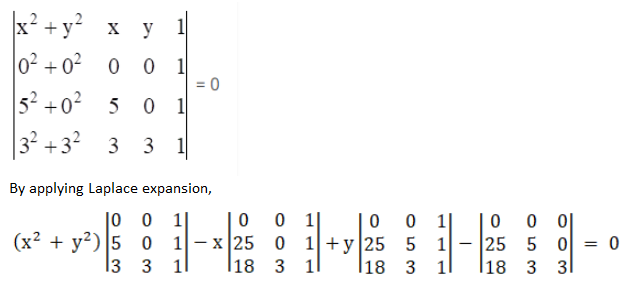⇒ 15(x2 + y2) – 75x – 15y = 0

⇒ 15(x2 + y2 – 5x – y) = 0

⇒ x2 + y2 – 5x – y = 0

By comparing with (i),

2g = -5 ⇒ g = -5/2

2f = -1 ⇒ f = -1/2

Centre = (-5/2, -1/2)

Radius = √(g2 + f2 – c)

= √[(-5/2)2 + (-1/2)2 – 0]

= √[(25/4) + (1/4)

= √[(25 + 1)/4]

= (√26)/ 2

SECTION C (20 Marks)

Question 19

(a) The average salary of male employees in an organization was Rs.420 and that of females was Rs.520. The mean salary of all the employees was Rs.500. Find the percentage of male and female employees. 

Solution:

Let x be the number of male and y be the number of female employees.

Given,

The average salary of male employees in an organization = Rs.420 and that of

The average salary of females = Rs.520.

The mean salary of all the employees = Rs.500

According to the given,

500 = (420x + 520y)/ (x + y)

500(x + y) = 420x + 520y

500x + 500y = 420x + 520y

500x – 420x = 520y – 500y

80x

= 20y

x/y = 20/80

x/y = ¼

Let x = k and y = 4k

Percentage of male employees = [k/ (k + 4k)] × 100 = (k/5k) × 100 = 20%

Percentage of female employees = 100 – 20 = 80%

(b) Calculate D4 and D6 for the following distribution: 

 C.I 10 – 20 20 – 30 30 – 40 40 – 50 50 – 60 60 – 70 Frequency 7 11 12 23 4 3

Solution:

 CI Frequency Cumulative frequency 10 – 20 7 7 20 – 30 11 18 30 – 40 12 30 40 – 50 23 53 50 – 60 4 57 60 – 70 3 60

Here,

N = 60

4N/10 = (4 × 60)/10 = 24Cumulative frequency greater than and nearest to 24 is 30 which lies in the interval 30 – 40.

l = 30

h = 10

f = 12

cf = 18

D4 = l + [{(4N/10) – cf}/ f] × h

= 30 + [(24 – 18)/ 12] × 10

= 30 + (60/12)

= 30 + 5

= 35

6N/10 = (6 × 60)/10 = 36

Cumulative frequency greater than and nearest to 36 is 53 which lies in the interval 40 – 50.

l = 40

h = 10

f = 23

cf = 30

D6 = l + [{(6N/10) – cf}/ f] × h

= 40 + [(36 – 30)/ 23] × 10

= 40 + (60/23)

= 40 + 2.61

= 42.61

OR

Calculate the mode for the following data:

 CI 0 – 10 10 – 20 20 – 30 30 – 40 40 – 50 50 – 60 60 – 70 70 – 80 Frequency 2 4 1 6 7 17 10 3

Solution:

From the given data,

Maximum frequency = 17

Modal class is 50 – 60.

Frequency of the modal class = f1 = 17

Frequency of the class preceding the modal class = f0 = 7

Frequency of the class succeeding the modal class = f2 = 10

Lower limit of the modal class = l = 50

Class height = h = 10

Mode = l + [(f1 – f0)/ (2f1 – f0 – f2)] × h

= 50 + [(17 – 7)/ (2 × 17 – 7 – 10)] × 10

= 50 + [10/ (34 – 17)] × 10

= 50 + (100/ 17)

= 50 + 5.88

= 55.88

Question 20

(a) Find the correlation coefficient of x and y when ∑x = 15, ∑y = 25, ∑xy = 83, ∑x2 = 55, ∑y2 = 135 and n = 5. 

Solution:

Given,

n = 5

∑x = 15

∑y = 25

∑x2 = 55

∑y2 = 135

∑xy = 83

Correlation coefficient is: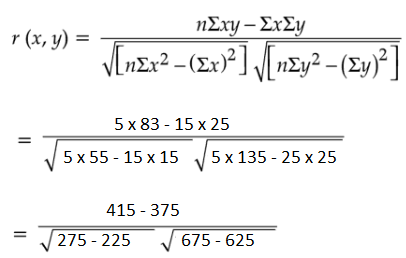= 40/ (√50 × √50)

= 40/50

= 0.8

(b) Find Karl Pearson’s Correlation Coefficient from the given data: 

 X 15 19 25 35 35 40 42 45 Y 30 35 36 40 42 48 55 80

Solution:

 x (x – mean) (x – 32) dx d2x y (y – mean) (y – 45.75) dy d2y dx dy 15 -17 289 30 -15.75 248.0625 267.75 19 -13 169 35 -10.75 115.5625 139.75 25 -7 49 36 -9.75 95.0625 68.25 35 3 9 40 -5.75 33.0625 -17.25 35 3 9 42 -3.75 14.0625 -11.25 40 8 64 48 2.25 5.0625 18 42 10 100 55 9.25 85.5625 92.5 45 13 169 80 34.25 1173.0625 445.25 ∑x = 256 ∑dx = 0 ∑d2x = 858 ∑y = 366 ∑dy = 0 ∑d2y = 1769.5 ∑dx dy = 1003

Mean of x = ∑x/n = 256/8 = 32

Mean of y = ∑y/n = 366/8 = 45.75

Karl Pearson’s Correlation Coefficient = (∑dx dy)/ (√∑d2x ∑d2y)

= 1003 / √(858 × 1769.5)

= 1003/ 1232.165

= 0.814

OR

Calculate the Spearman’s rank correlation coefficient for the following data:

 X 35 54 80 95 73 73 35 91 83 81 Y 70 75 95 38 75 70 90 75 60 40

Solution:

 X Rank (R1) Y Rank (R2) d = R1 – R2 d2 35 9.5 70 6.5 3 9 54 8 75 4 4 16 80 5 95 1 4 16 95 1 38 10 -9 81 73 6.5 75 4 2.5 6.25 73 6.5 70 6.5 0 0 35 9.5 90 2 7.5 56.25 91 2 75 4 -2 4 83 3 60 8 -5 25 81 4 40 9 -5 25

n = 10

∑d2 = 238.5

The spearman’s rank correlation coefficient = 1 – [(6 × ∑d2)/ n(n2 – 1)]

= 1 – [(6 × 238.5)/ 10(100 – 1)]

= 1 – [1431/ 10 × 99]

= 1 – (1431/990)

= (990 – 1431)/ 990

= -441/990

= -0.445

Question 21 

Find the consumer price index for 2014 on the basis of 2010 from the following data using a weighted average of price relative method:

 Commodity 2010 2014 Weight A 20 40 8 B 50 60 10 C 40 50 14 D 20 20 19

Solution:

 Commodity Weights (w) Year 2010 (p0) Year 2014 (p1) Price relative I = (p1/p0) × 100 Iw A 8 20 40 200 1600 B 10 50 60 120 1200 C 14 40 50 125 1750 D 19 20 20 100 1900 Total ∑w = 51 ∑Iw = 6450

Index number of weighted average of price relatives = ∑Iw/ ∑w

= 6450/ 51

= 126.47

Question 22 

Construct a 3 yearly moving average from the following data and represent it using a graph.

 Year 1994 1995 1996 1997 1998 1999 2000 2001 2002 2003 Sales 18 22 20 26 30 22 24 28 32 35

Solution:

 Year Sales 3 days moving average 1994 18 1995 22 (18 + 22 + 20)/3 = 60/3 = 20 1996 20 (22 + 20 + 26)/ 3 = 68/3 = 22.67 1997 26 (20 + 26 + 30)/3 = 76/3 = 25.33 1998 30 (26 + 30 + 22)/3 = 78/3 = 26 1999 22 (30 + 22 + 24)/3 = 76/3 = 25.33 2000 24 (22 + 24 + 28)/3 = 74/3 = 24.67 2001 28 (24 + 28 + 32)/3 = 84/3 = 28 2002 32 (28 + 32 + 35)/3 = 75/3 = 25 2003 35

Graph: